# Selina Solutions Concise Physics Class 10 Chapter 7 Sound

Selina Solutions Concise Physics Class 10 Chapter 7 Sound has been solved by expert teachers having vast knowledge in Physics. Students who practice these solutions come out with good marks in the exams, as the solutions are crafted in simple language. Those who are unable to understand the chapter during class hours can follow these solutions to overcome this issue.

Selina Solutions Concise Physics Class 10 plays a vital role in clearing students’ doubts easily. Students who find obstacles in doing their homework can use solutions to answer more effectively. These solutions are very helpful in revising the chapter while preparing for the examination. Solutions designed by experts help students to improve their skills, which are important from an exam point of view. Download the Selina Solutions Concise Physics Class 10 Chapter 7 Sound from the links given below

## Selina Solutions Concise Physics Chapter 7 Sound:-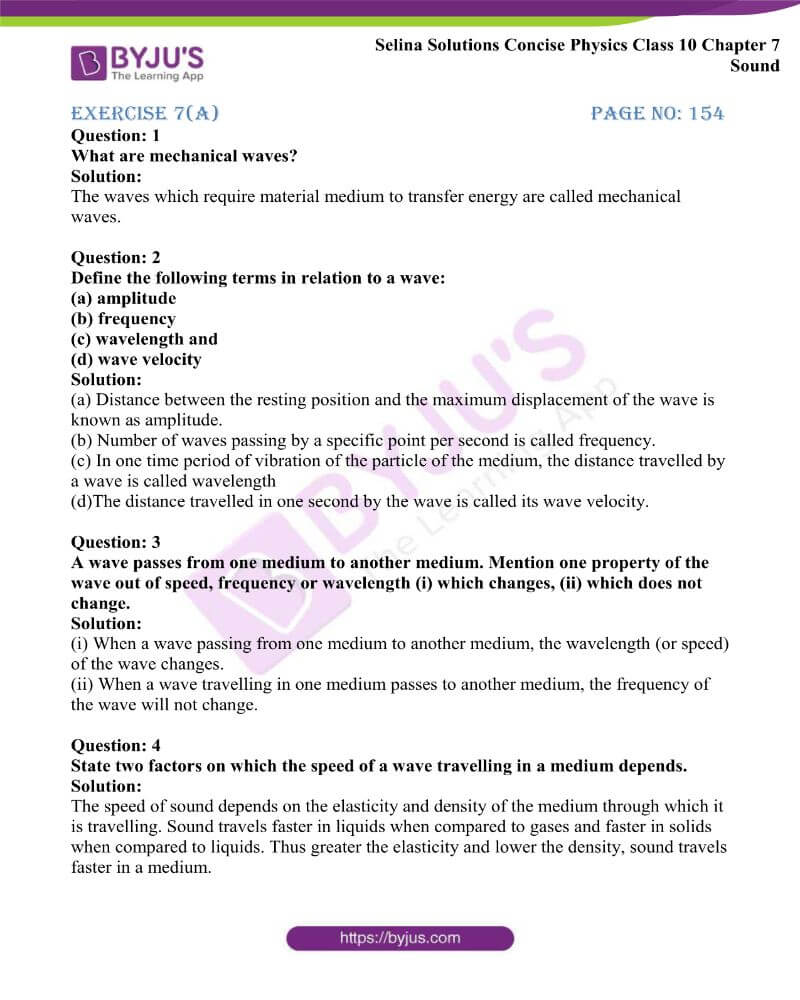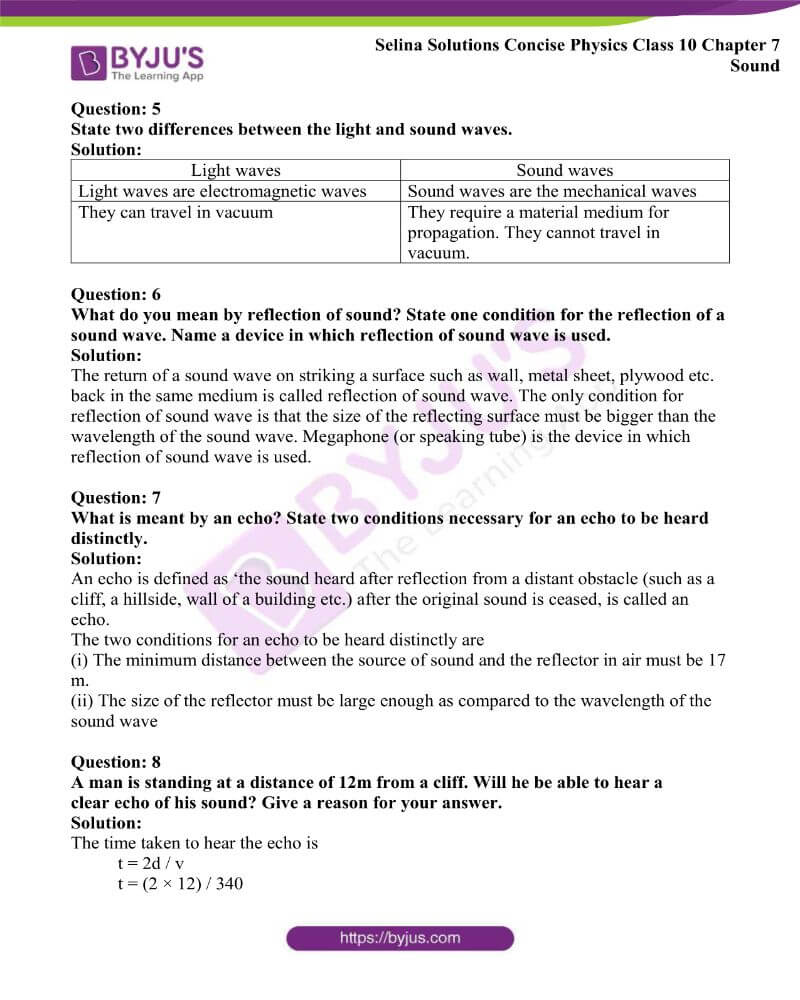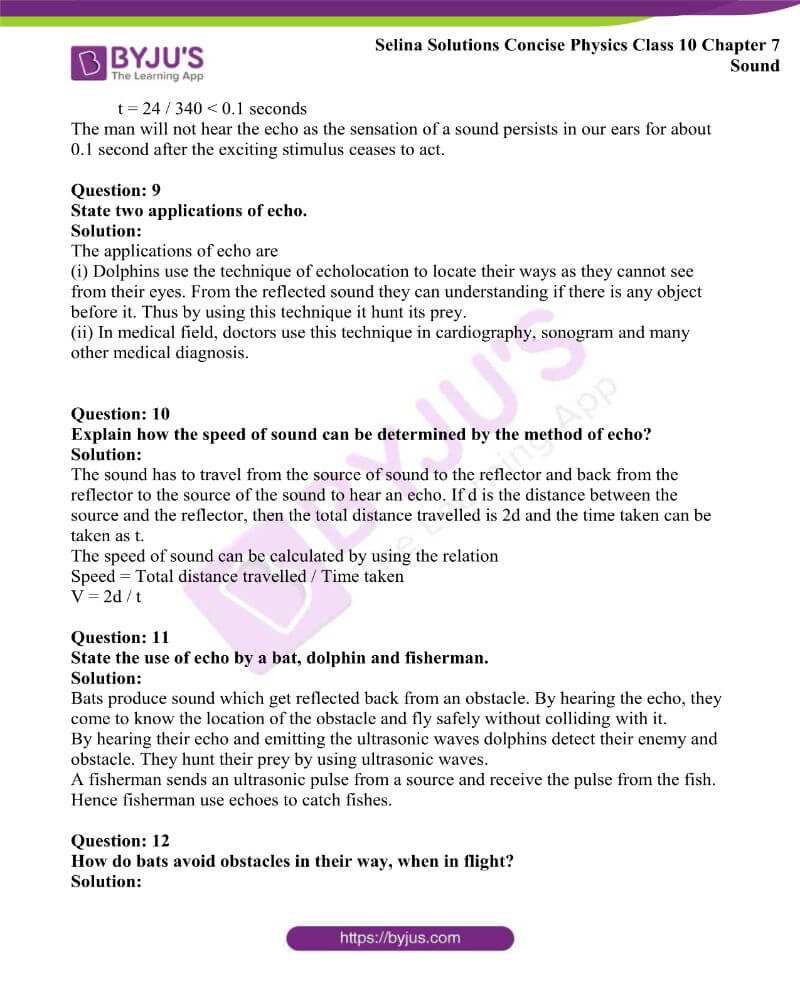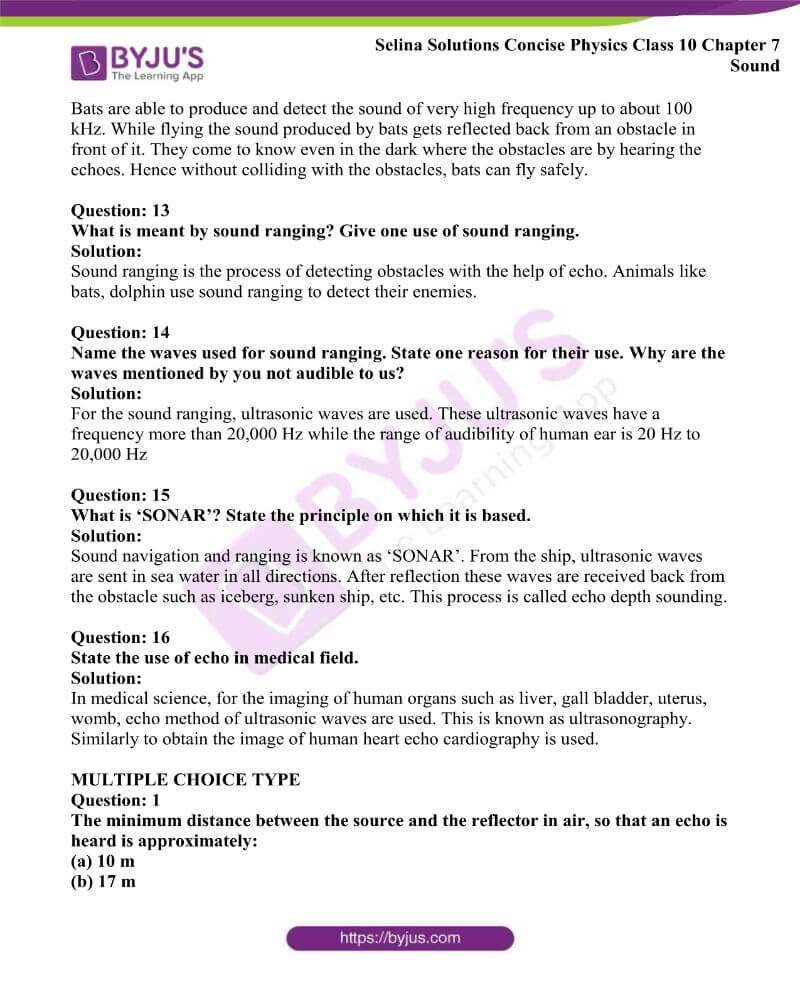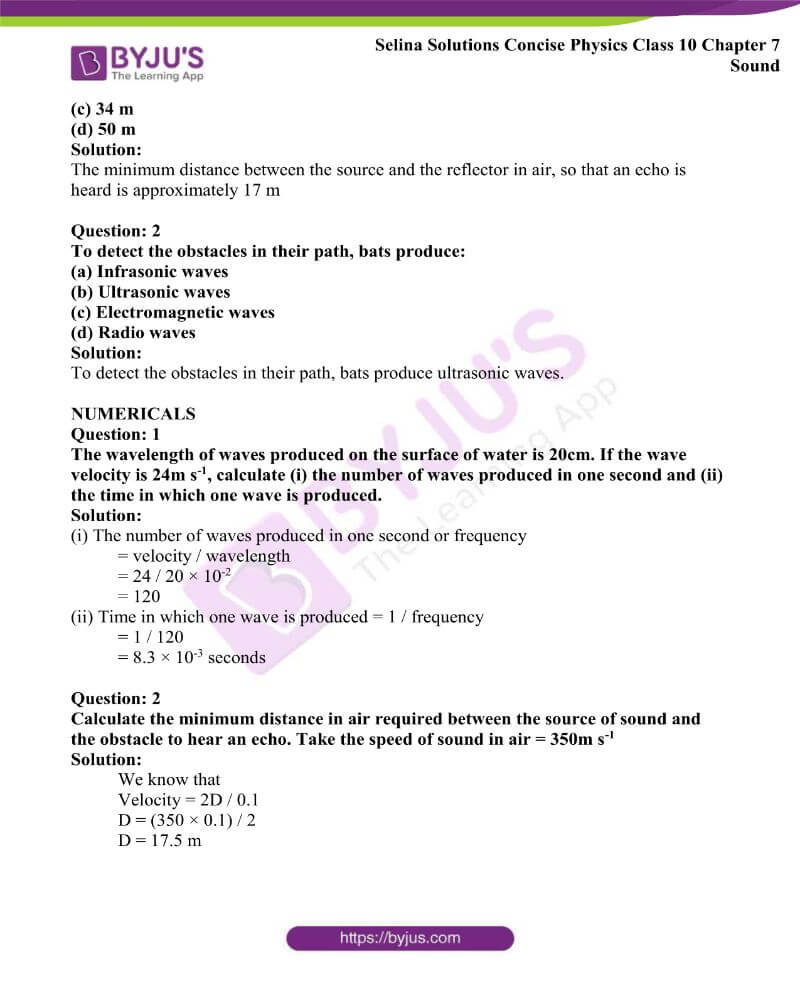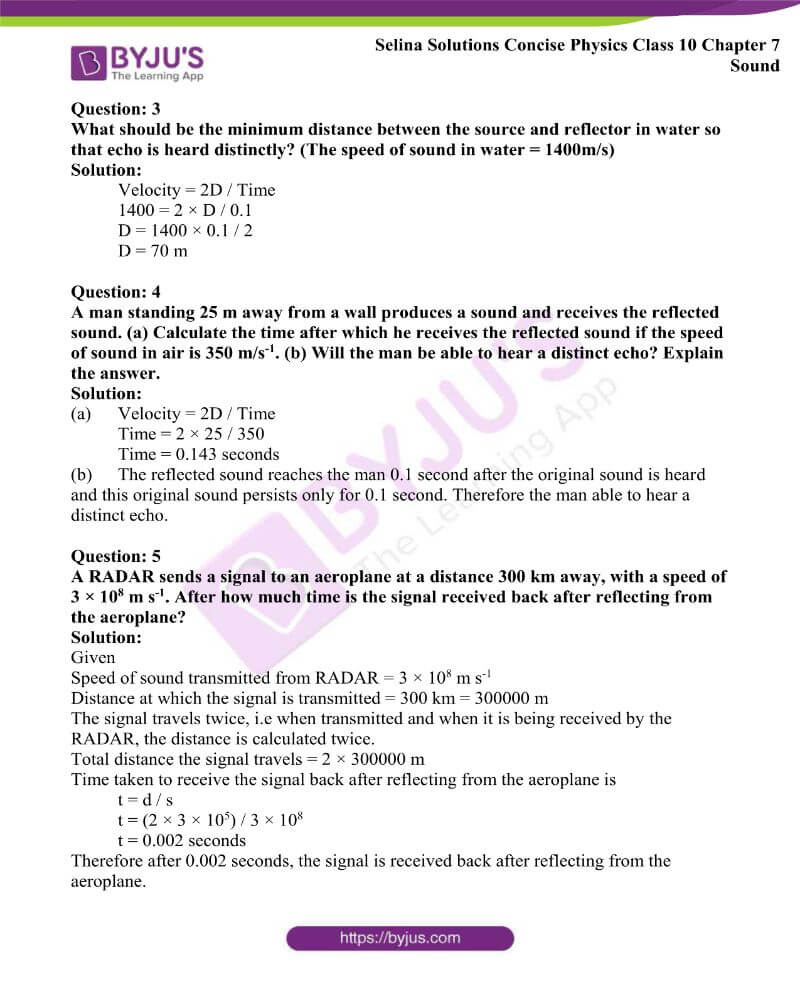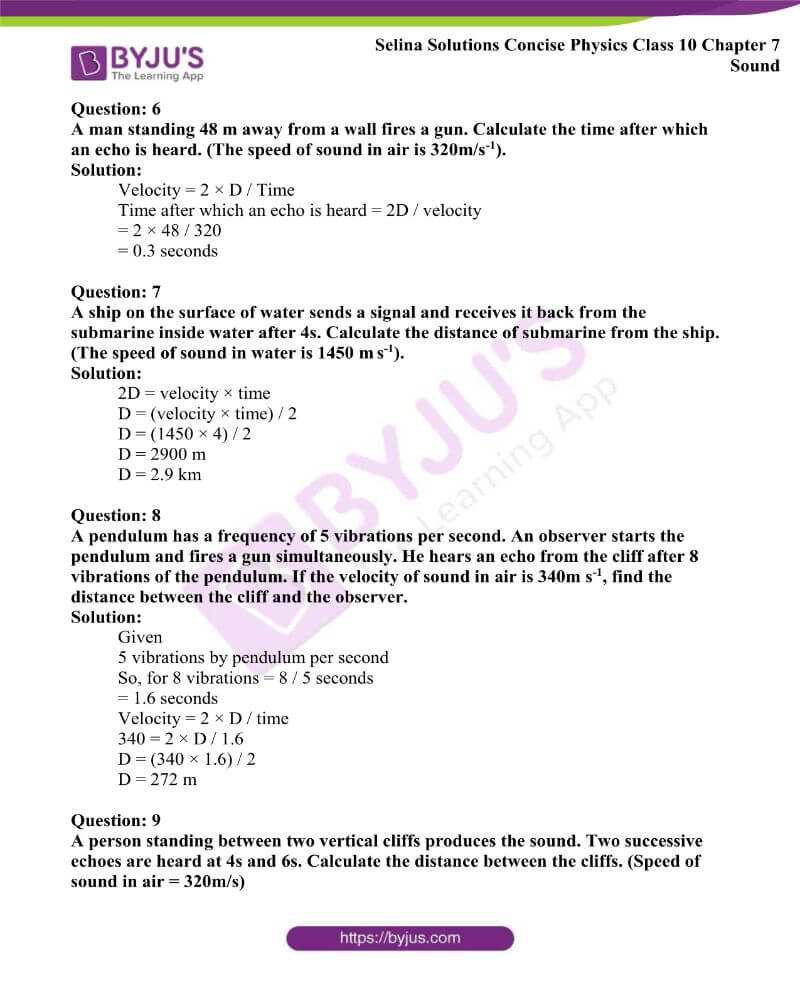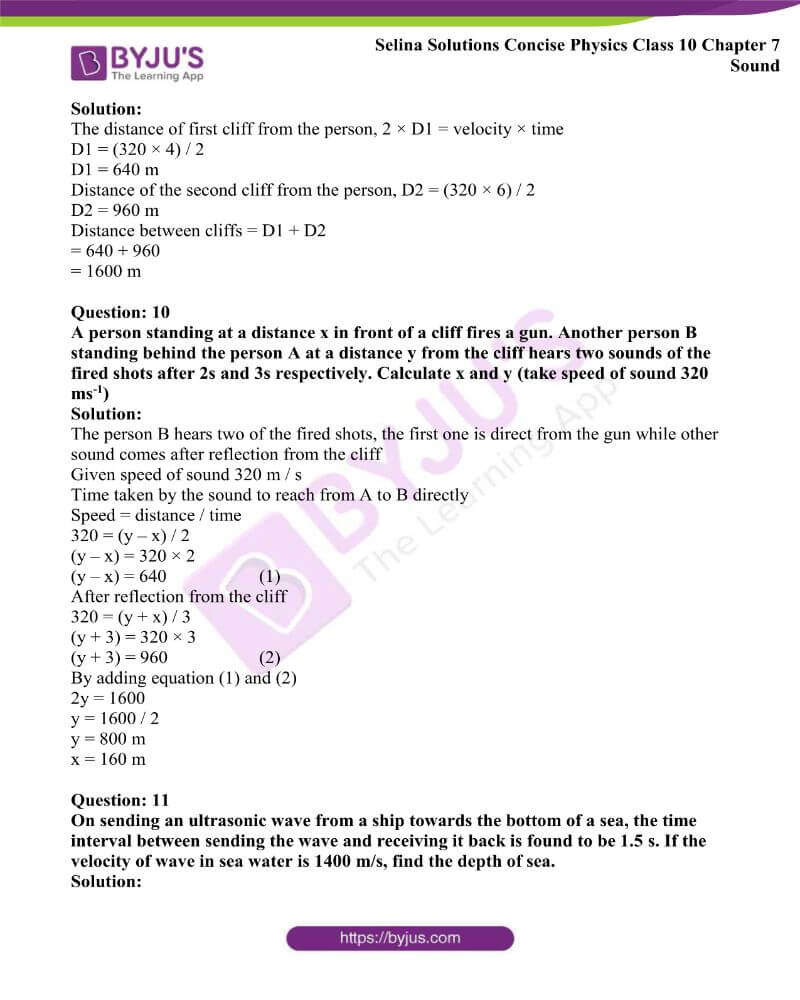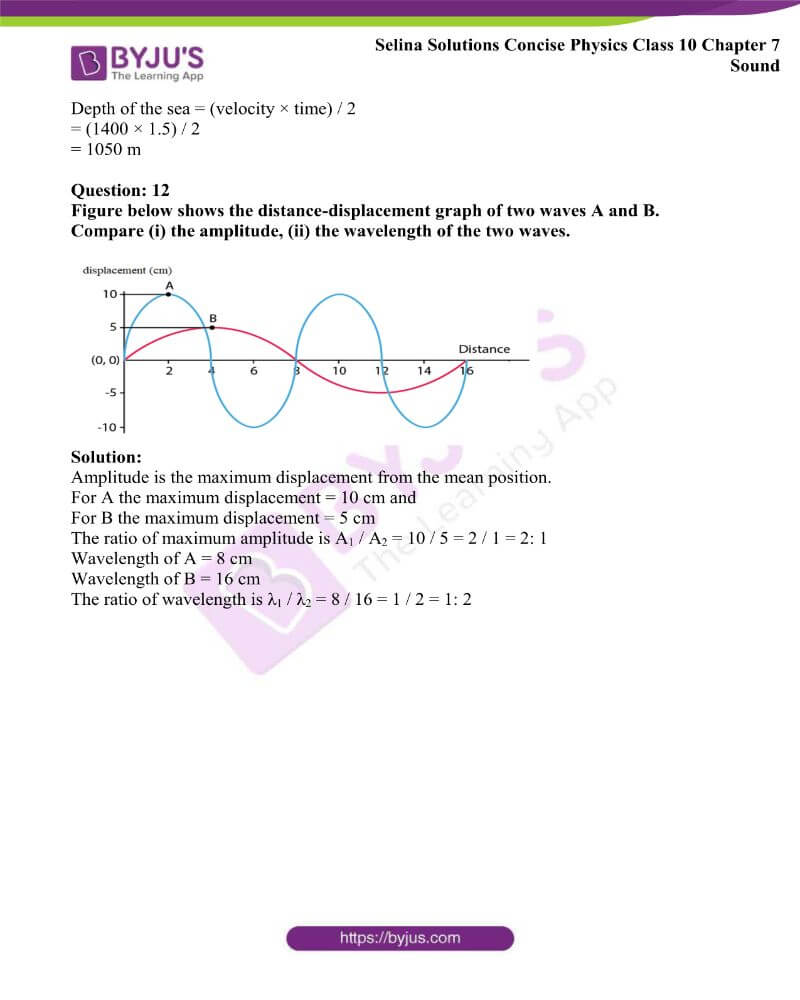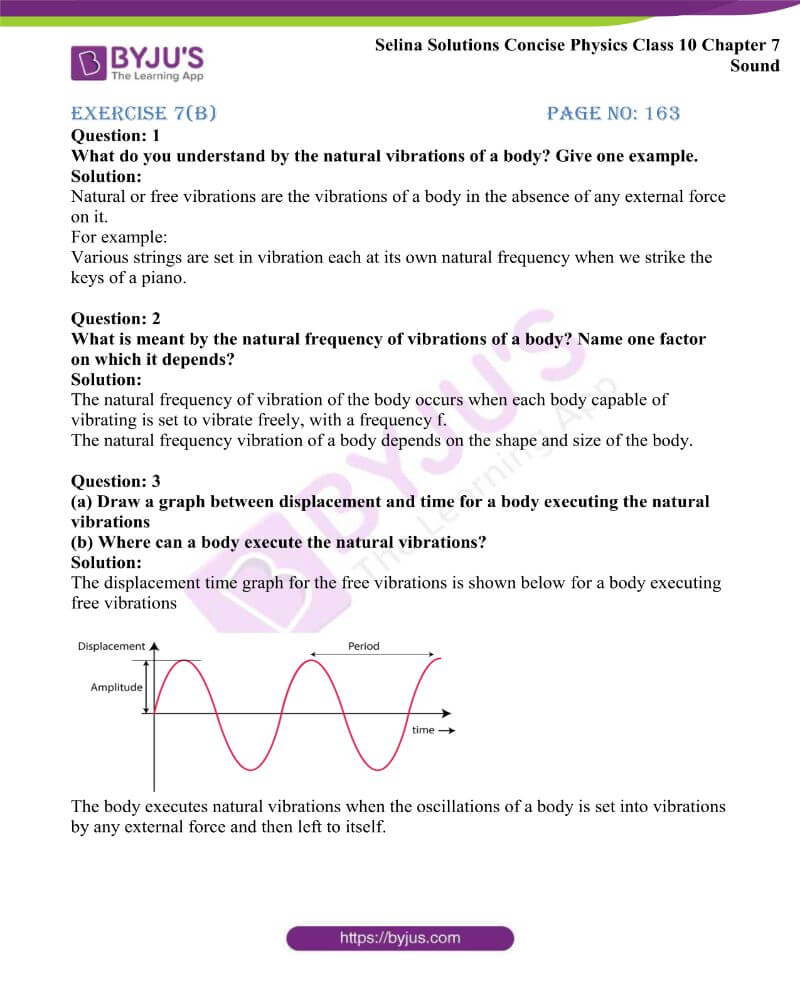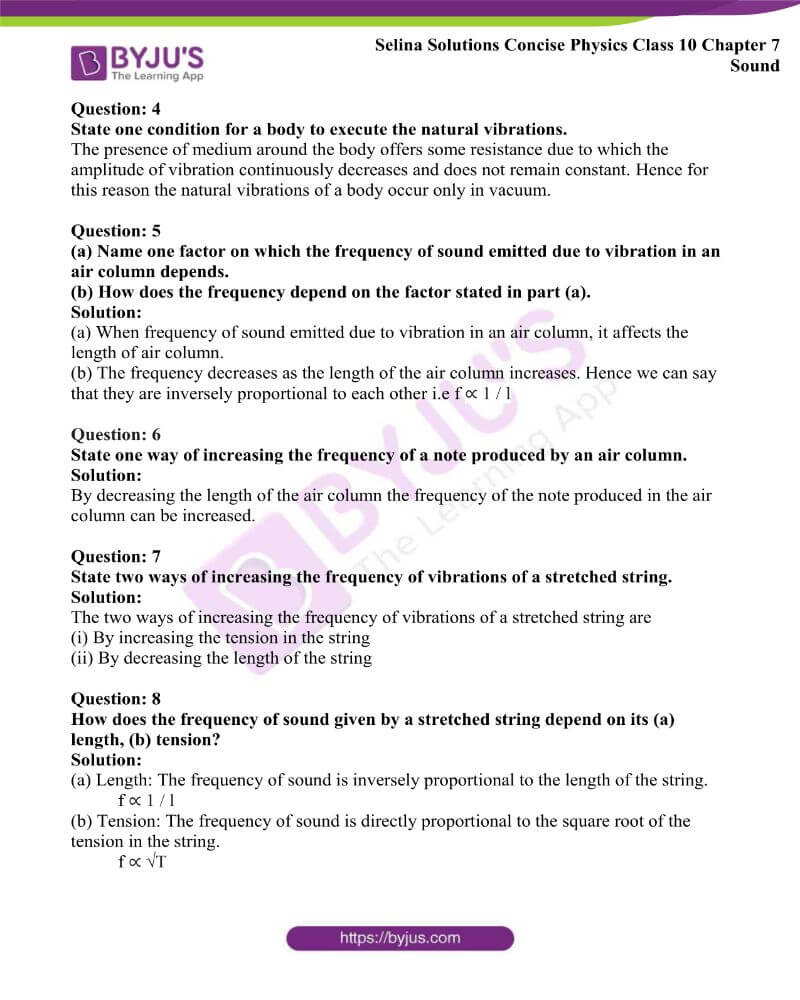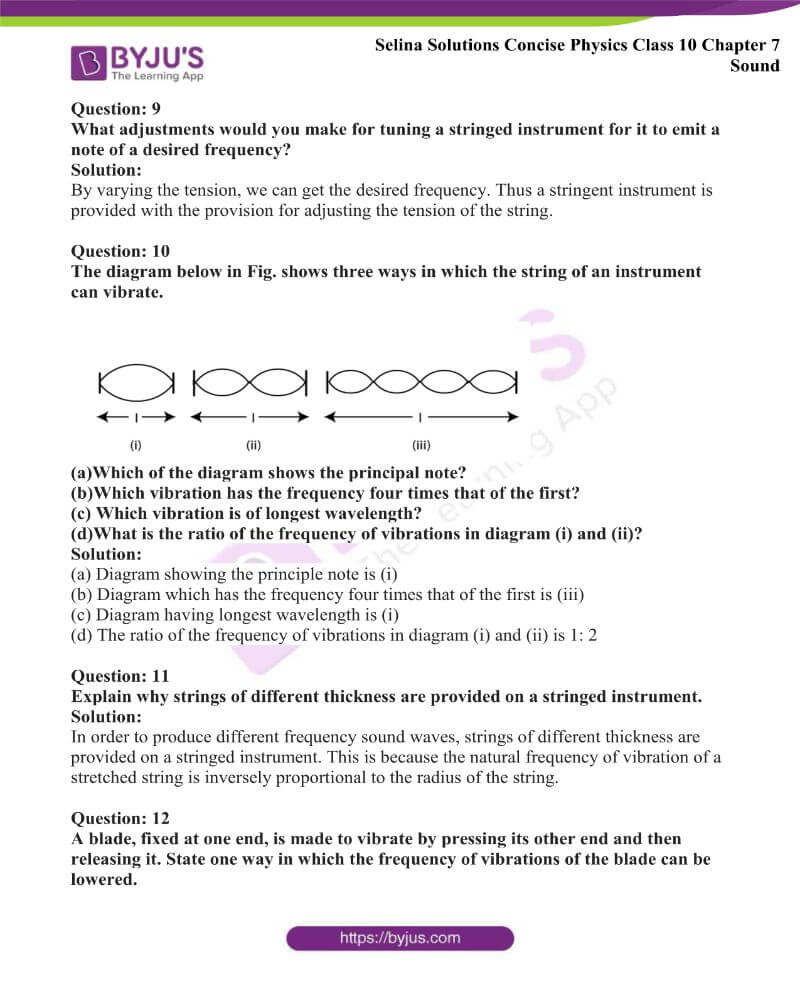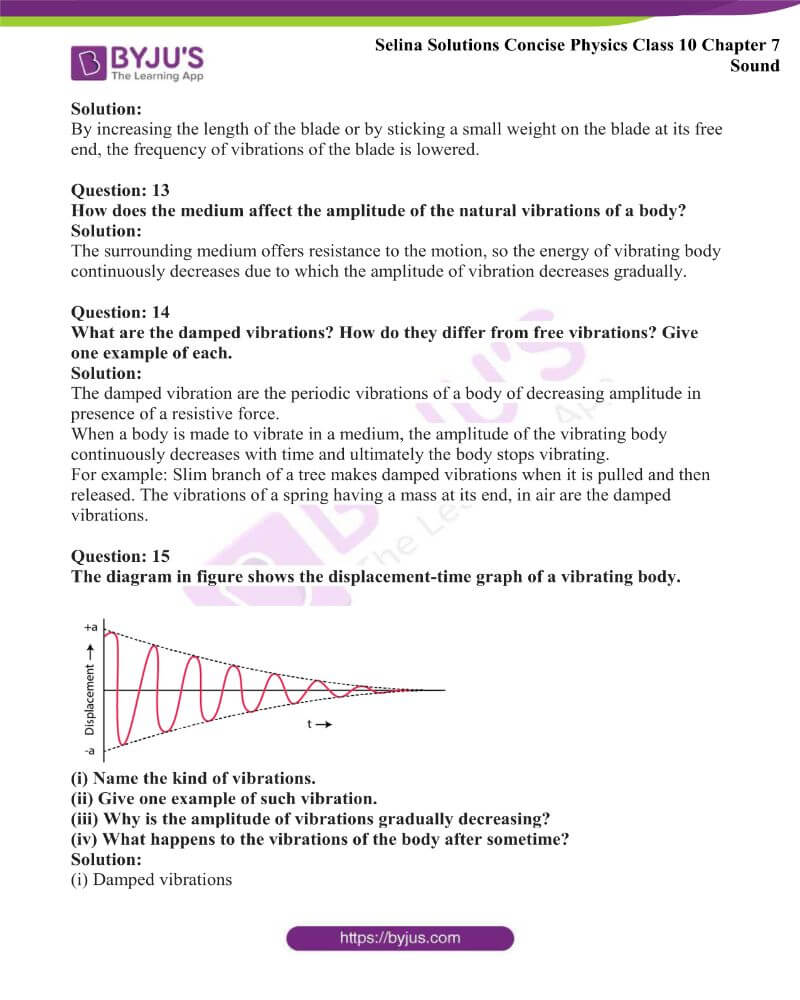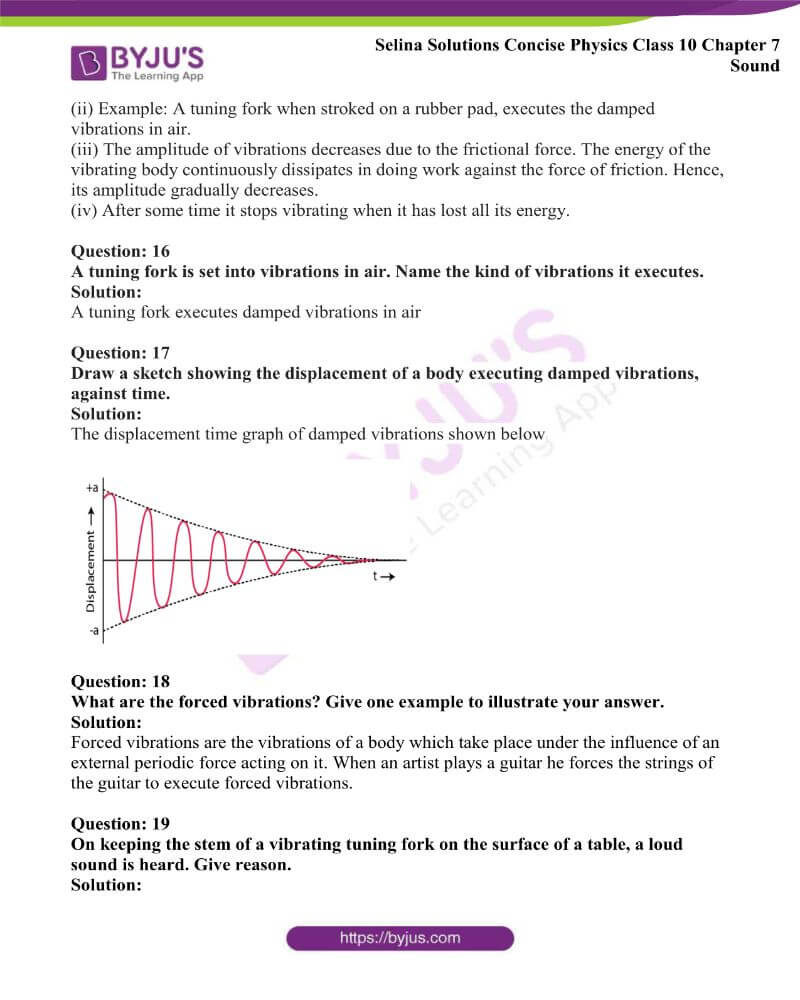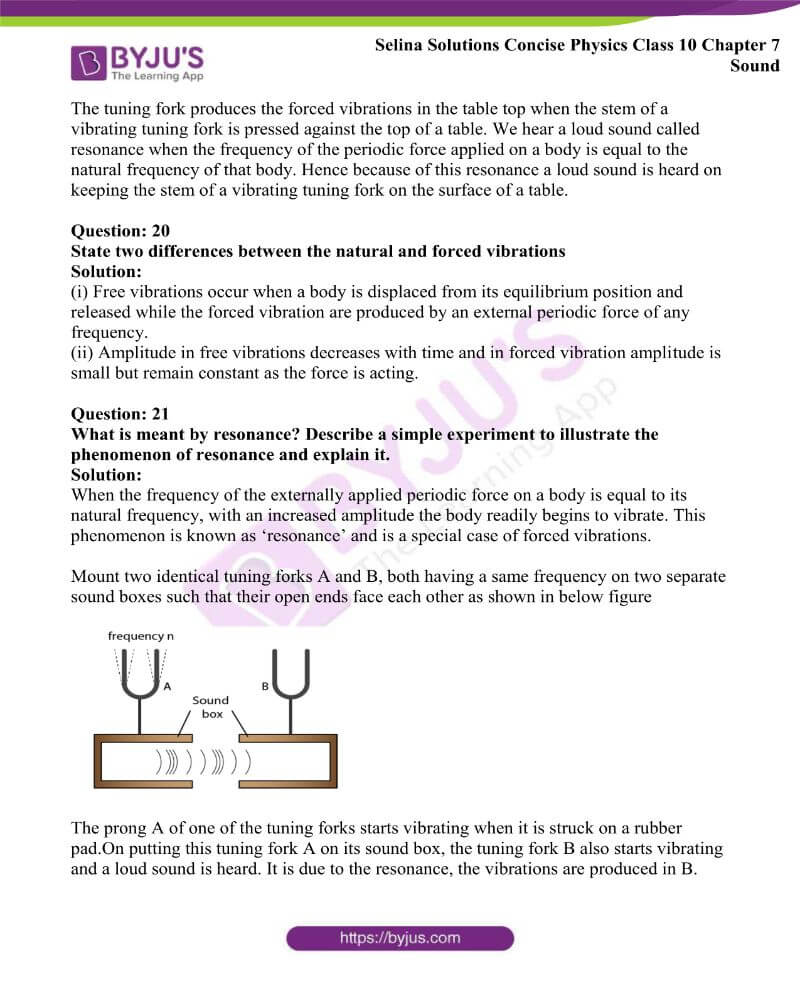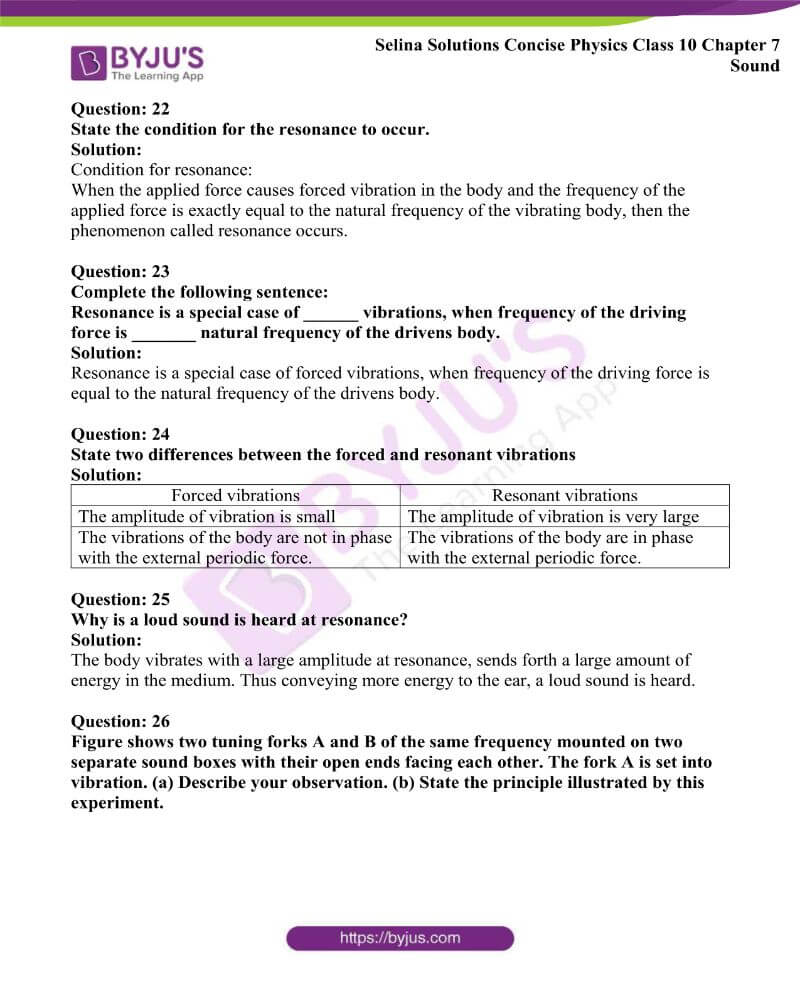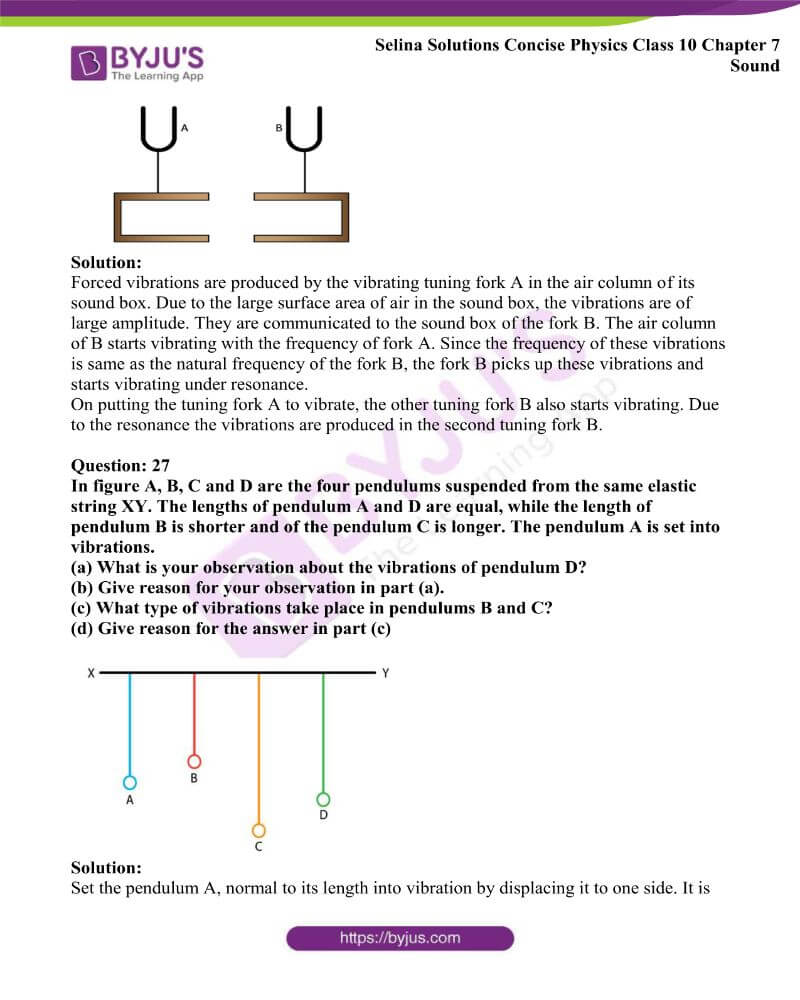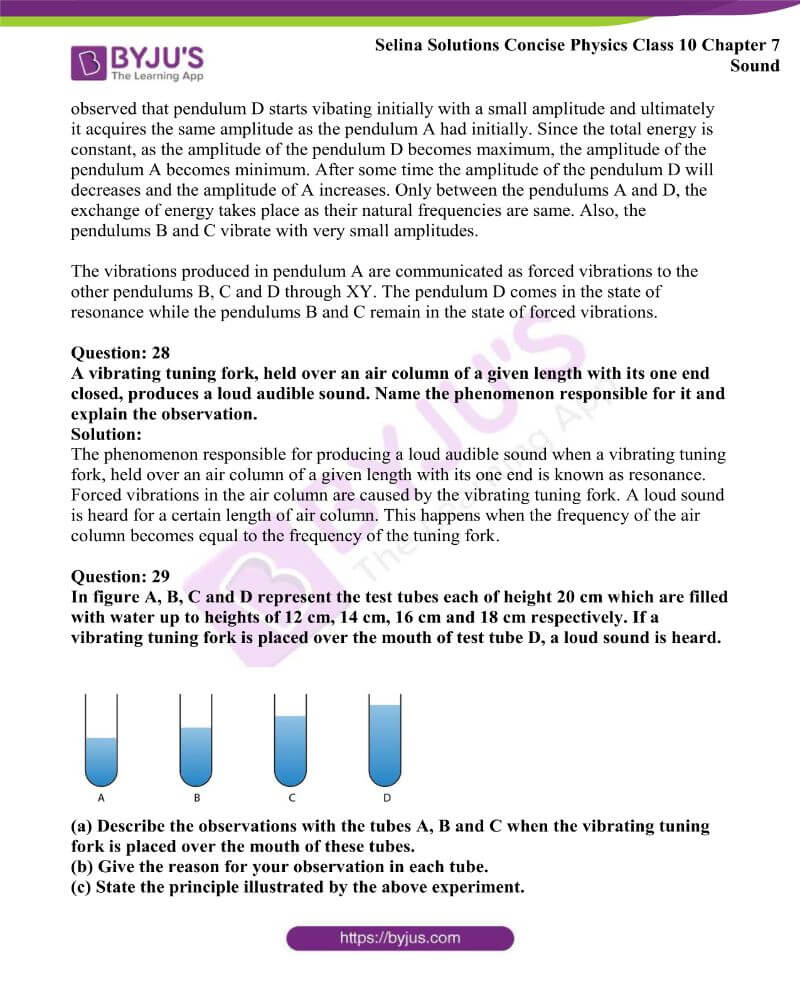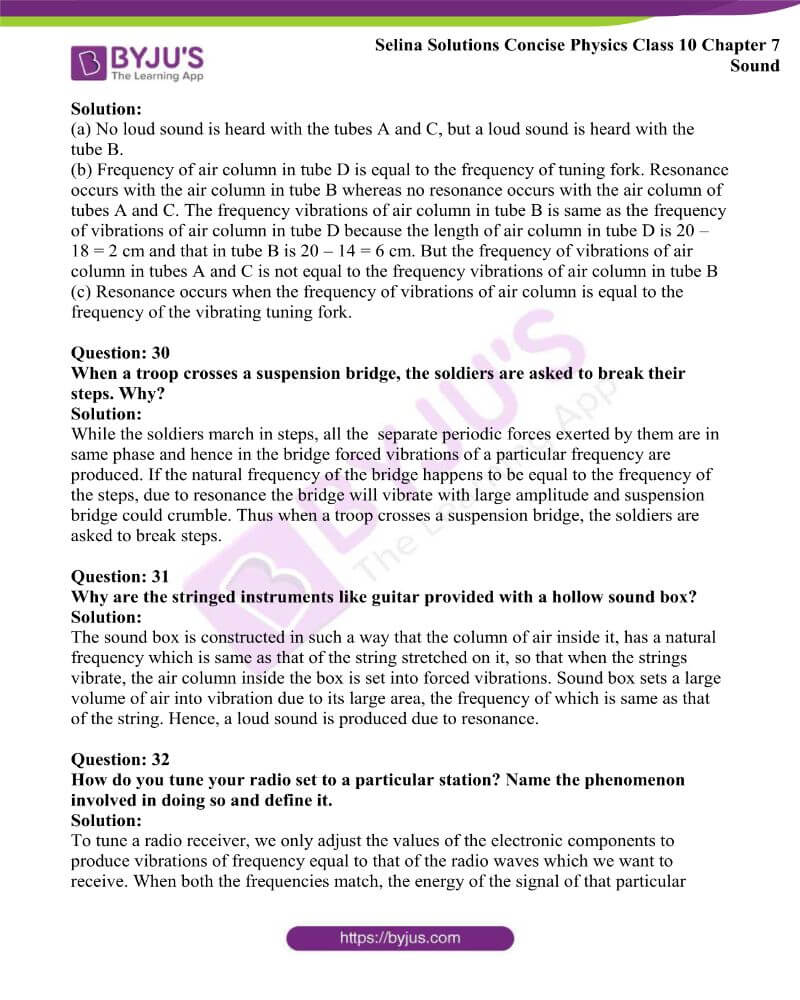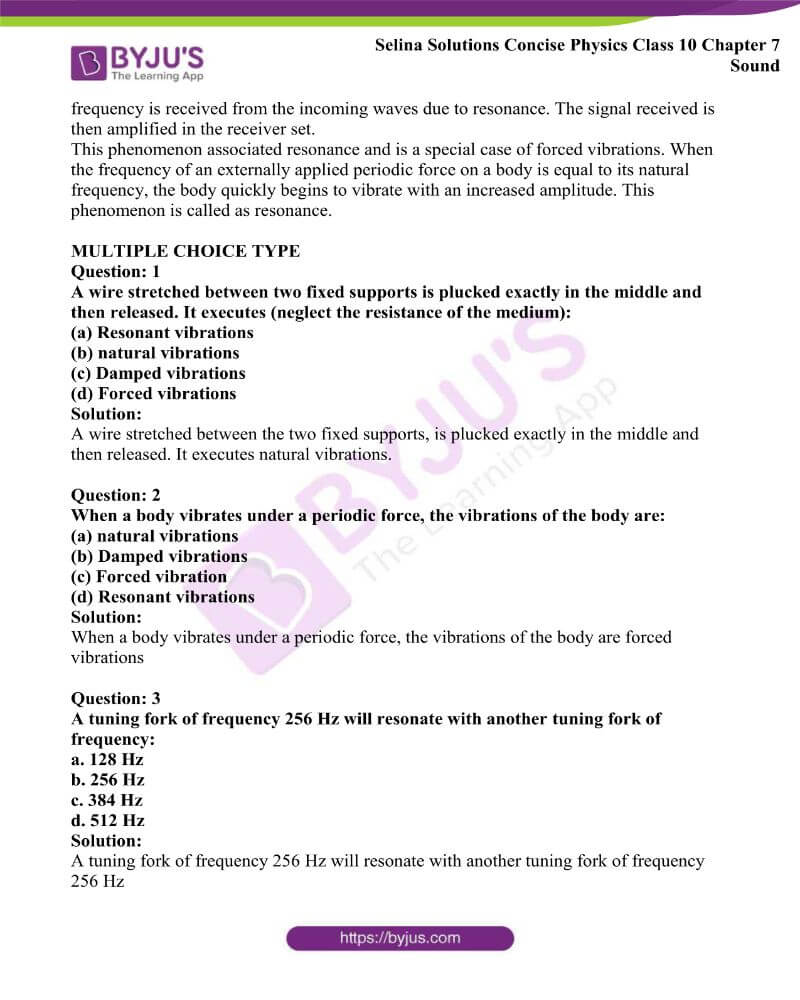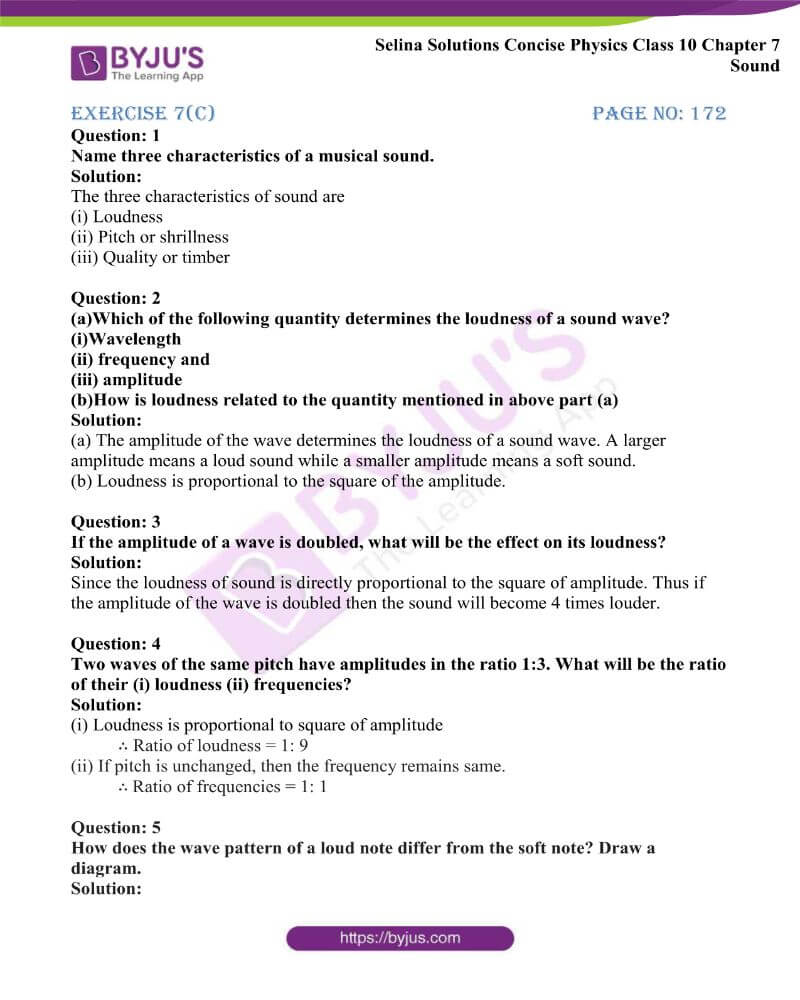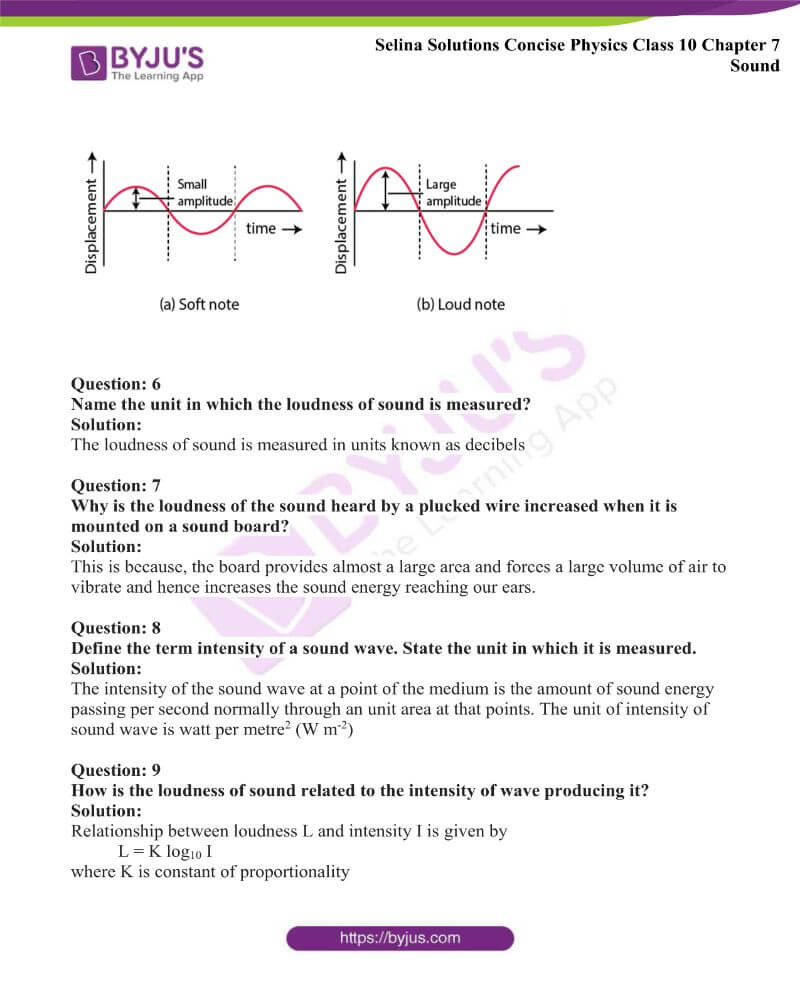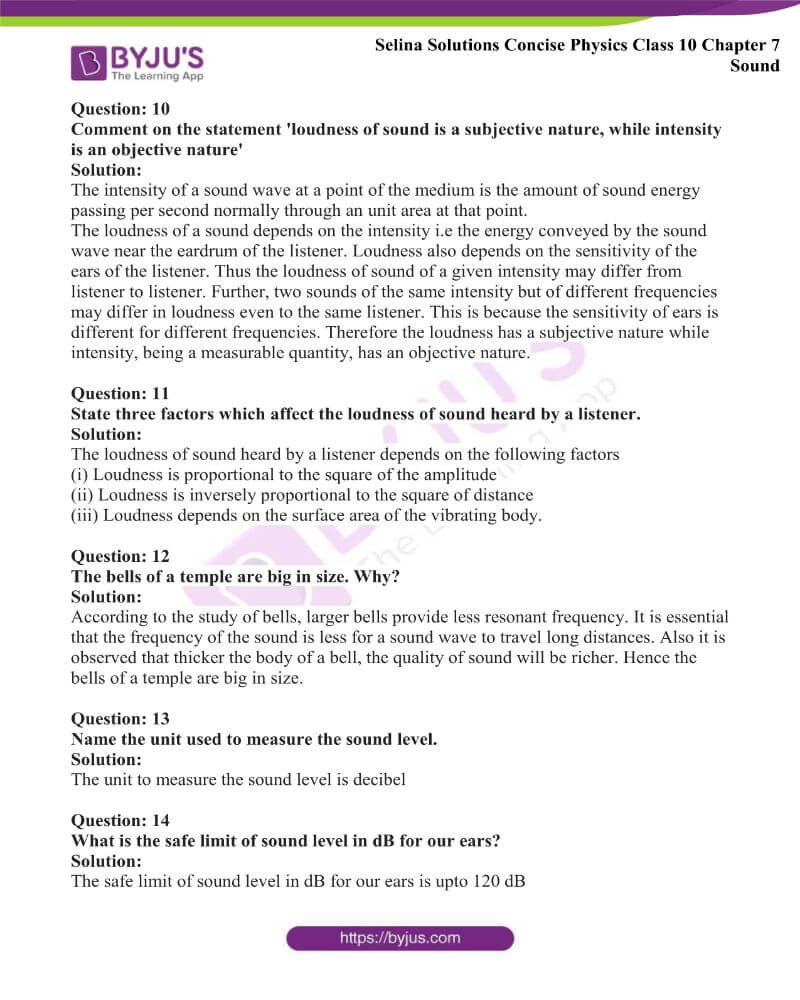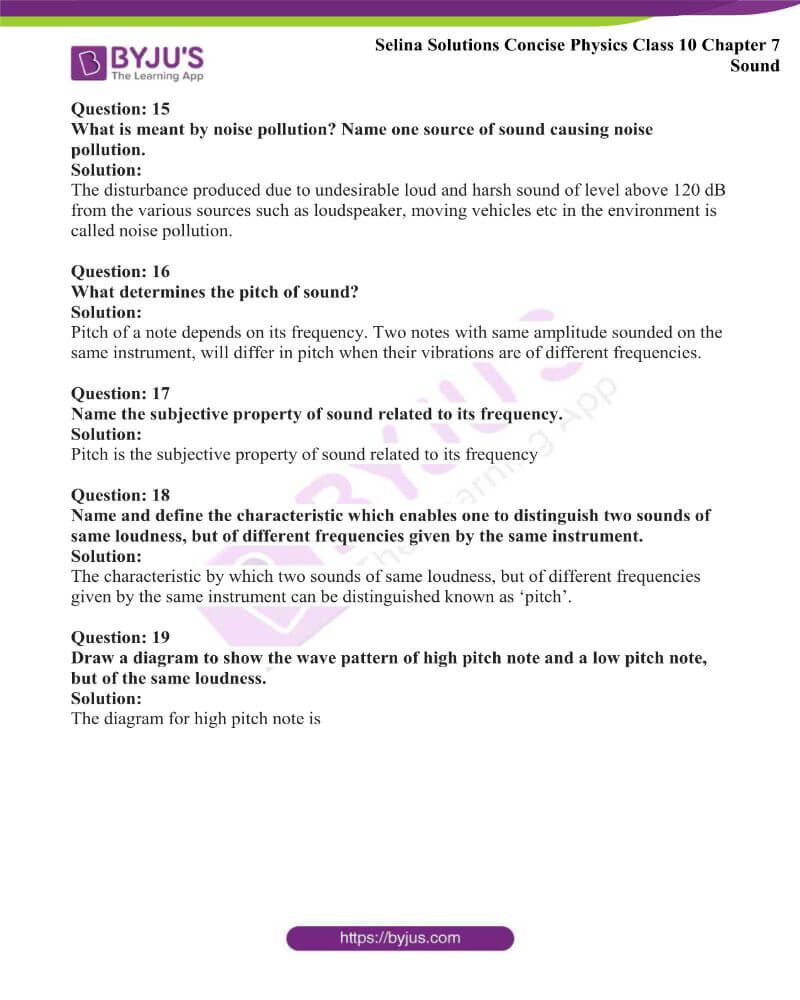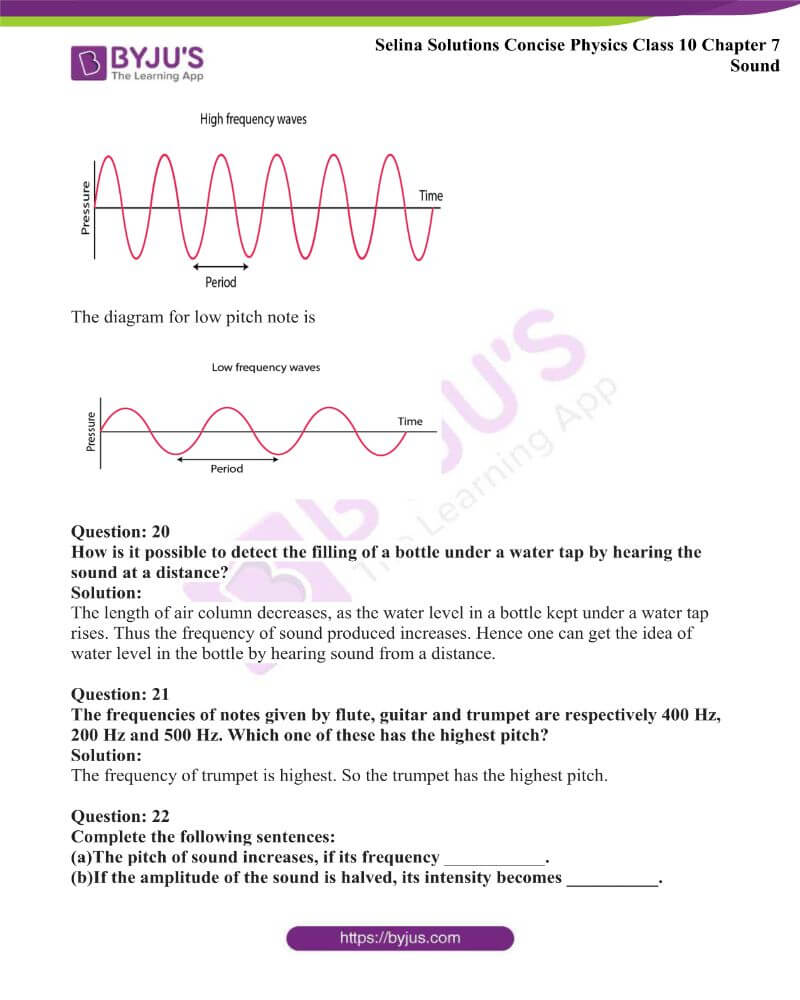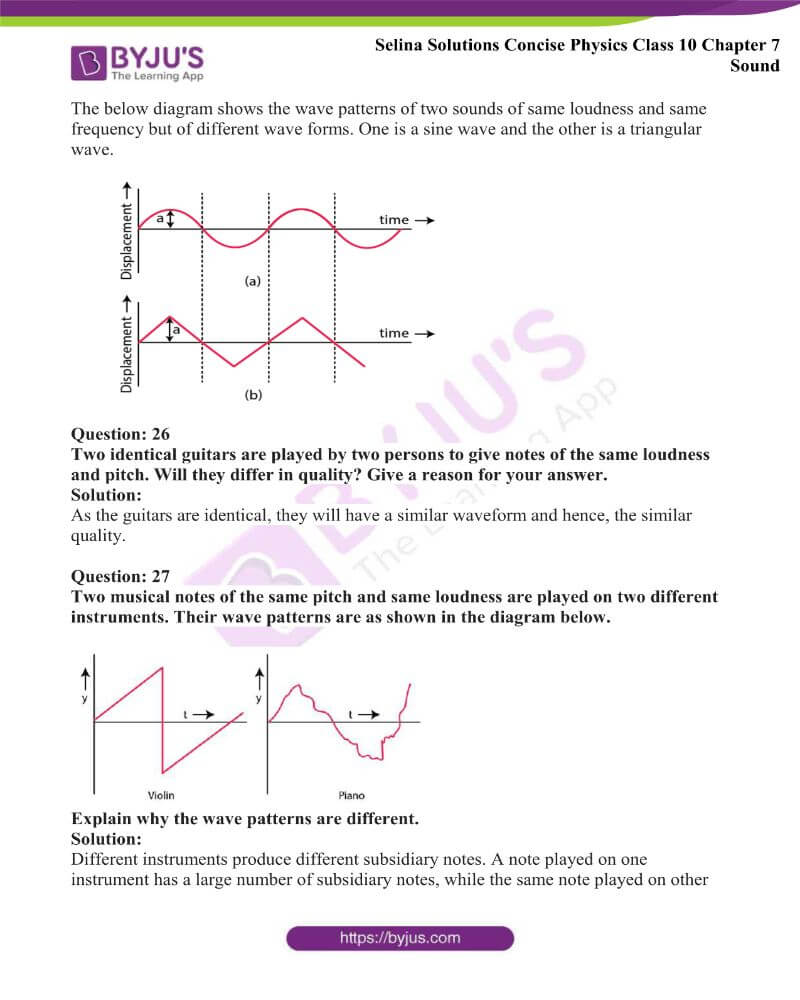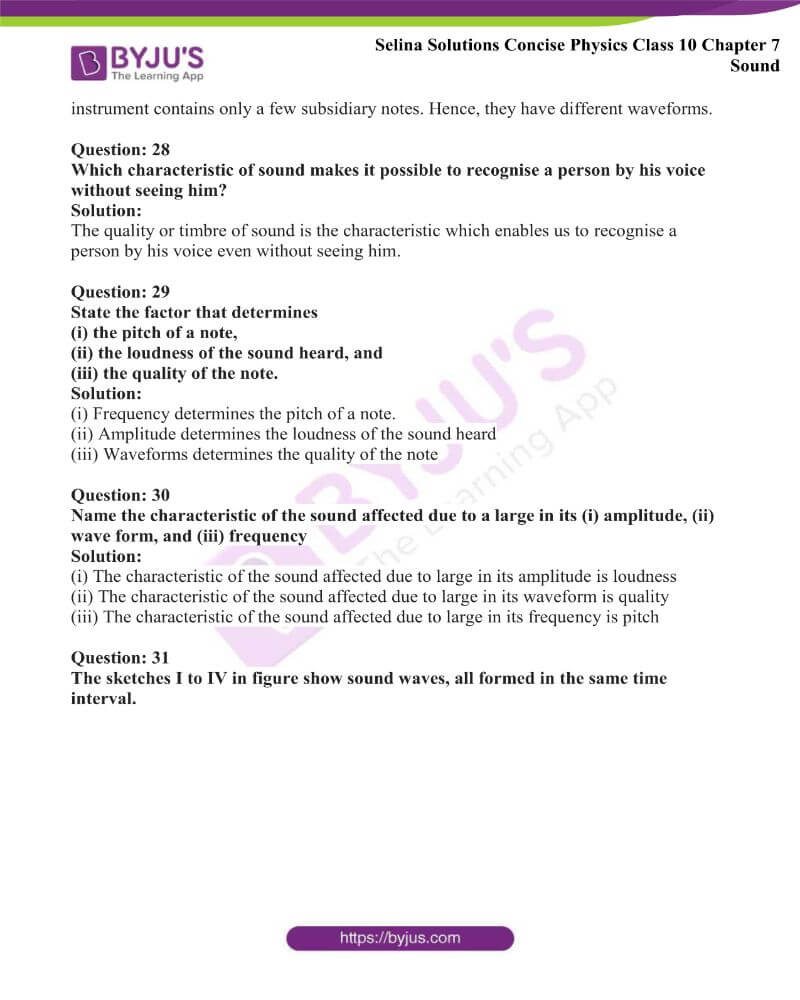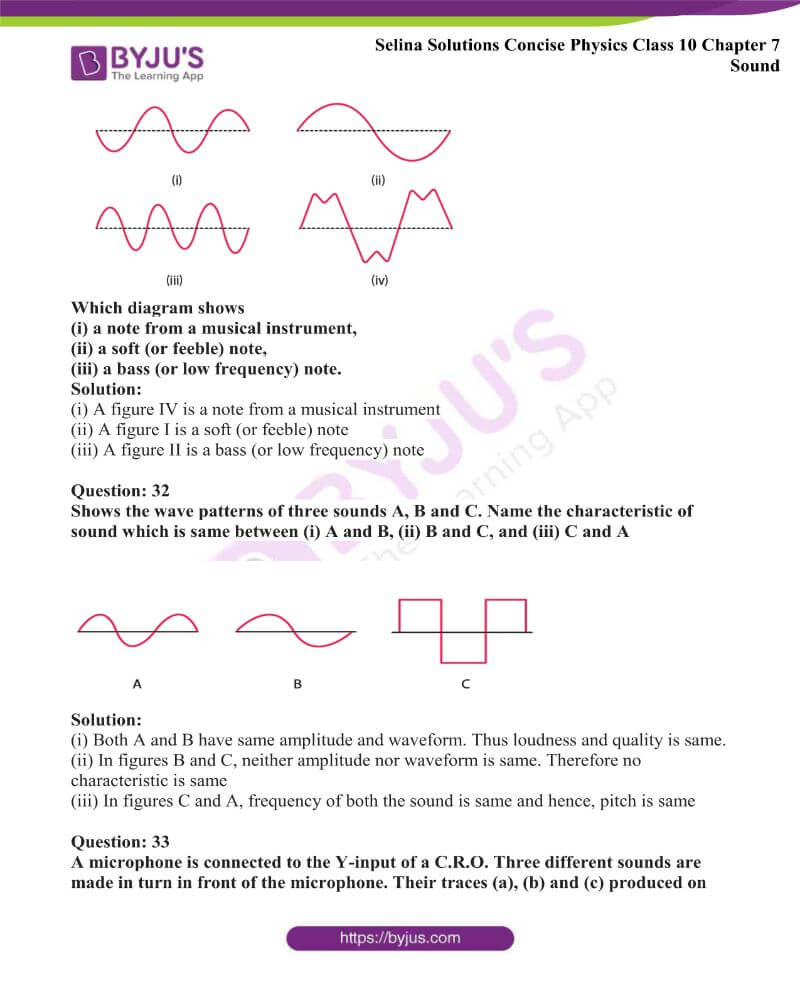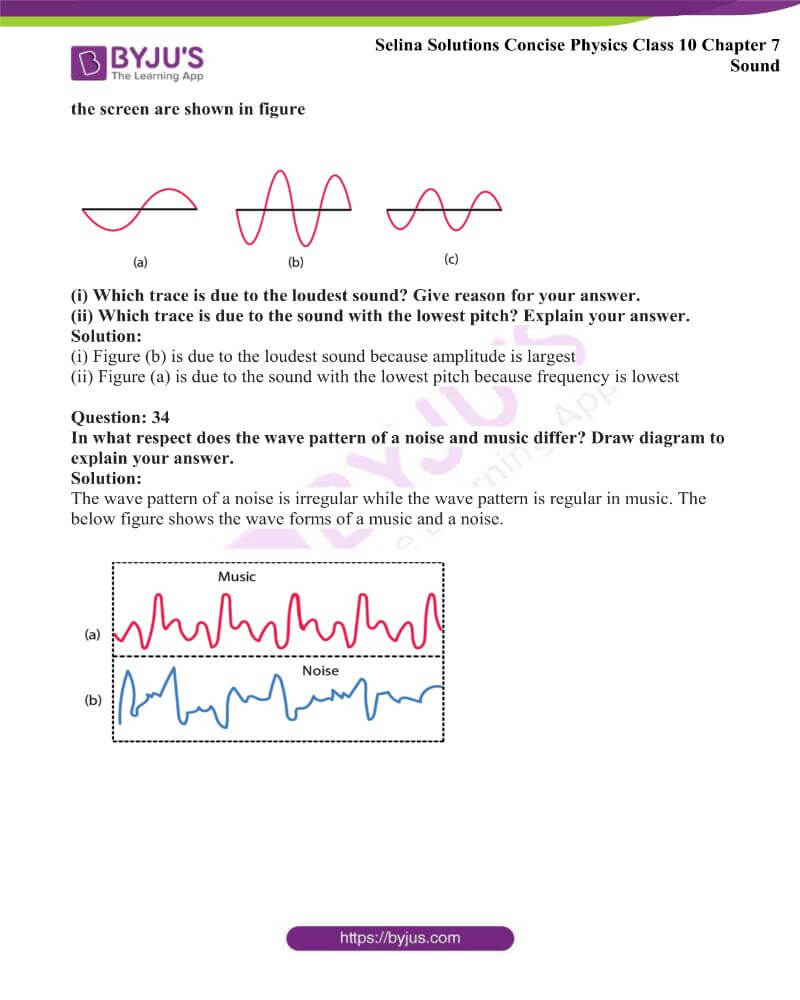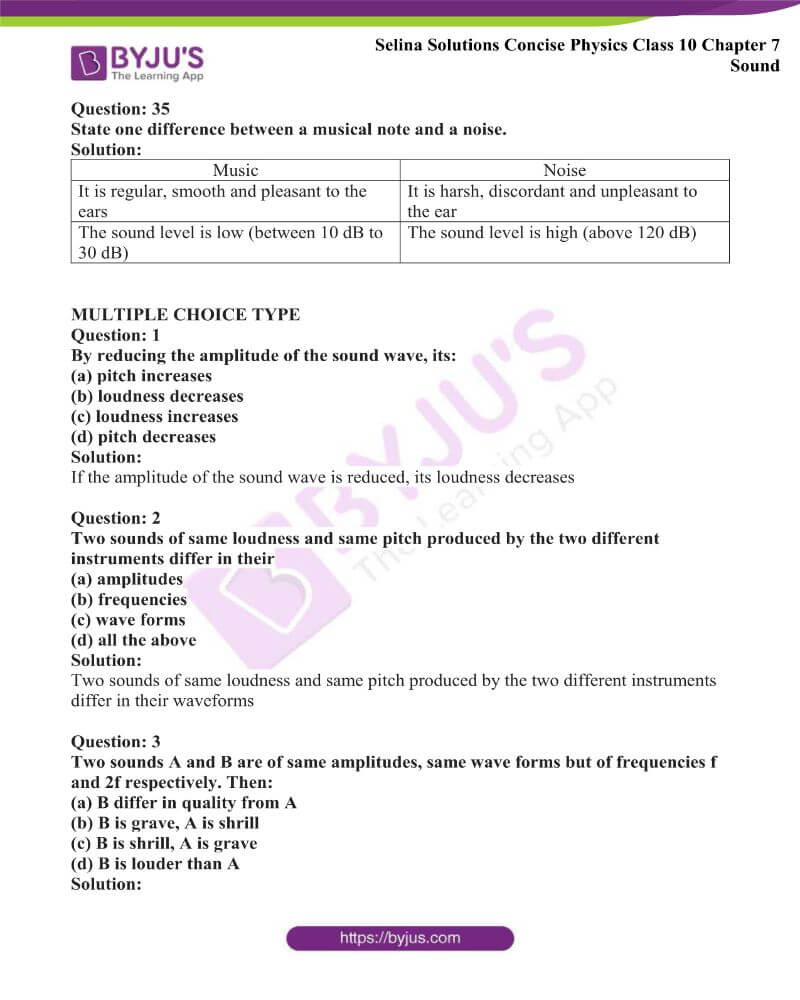### Access Answers to Physics Selina Solutions Concise Physics Class 10 Chapter 7 Sound

Exercise 7(a) page no: 154

Question: 1

What are mechanical waves?

Solution:

The waves which require material medium to transfer energy are called mechanical waves.

Question: 2

Define the following terms in relation to a wave:

(a) amplitude

(b) frequency

(c) wavelength and

(d) wave velocity

Solution:

(a) Distance between the resting position and the maximum displacement of the wave is known as amplitude.

(b) Number of waves passing by a specific point per second is called frequency.

(c) In one time period of vibration of the particle of the medium, the distance travelled by a wave is called wavelength

(d)The distance travelled in one second by the wave is called its wave velocity.

Question: 3

A wave passes from one medium to another medium. Mention one property of the wave out of speed, frequency or wavelength (i) which changes, (ii) which does not change.

Solution:

(i) When a wave passing from one medium to another medium, the wavelength (or speed) of the wave changes.

(ii) When a wave travelling in one medium passes to another medium, the frequency of the wave will not change.

Question: 4

State two factors on which the speed of a wave travelling in a medium depends.

Solution:

The speed of sound depends on the elasticity and density of the medium through which it is travelling. Sound travels faster in liquids when compared to gases and faster in solids when compared to liquids. Thus greater the elasticity and lower the density, sound travels faster in a medium.

Question: 5

State two differences between the light and sound waves.

Solution:

 Light waves Sound waves Light waves are electromagnetic waves Sound waves are the mechanical waves They can travel in a vacuum They require a material medium for propagation. They cannot travel in a vacuum.

Question: 6

What do you mean by reflection of sound? State one condition for the reflection of a sound wave. Name a device in which reflection of a sound wave is used.

Solution:

The return of a sound wave on striking a surface such as a wall, metal sheet, plywood etc. back in the same medium is called reflection of the sound wave. The only condition for reflection of a sound wave is that the size of the reflecting surface must be bigger than the wavelength of the sound wave. Megaphone (or speaking tube) is the device in which reflection of a sound wave is used.

Question: 7

What is meant by an echo? State two conditions necessary for an echo to be heard distinctly.

Solution:

An echo is defined as ‘the sound heard after reflection from a distant obstacle (such as a cliff, a hillside, the wall of a building etc.) after the original sound is ceased, is called an echo.

The two conditions for an echo to be heard distinctly are

(i) The minimum distance between the source of sound and the reflector in the air must be 17 m.

(ii) The size of the reflector must be large enough as compared to the wavelength of the sound wave

Question: 8

A man is standing at a distance of 12m from a cliff. Will he be able to hear a clear echo of his sound? Give a reason for your answer.

Solution:

The time taken to hear the echo is

t = 2d / v

t = (2 × 12) / 340

t = 24 / 340 < 0.1 seconds

The man will not hear the echo as the sensation of a sound persists in our ears for about 0.1 second after the exciting stimulus ceases to act.

Question: 9

State two applications of echo.

Solution:

The applications of echo are

(i) Dolphins use the technique of echolocation to locate their ways as they cannot see from their eyes. From the reflected sound they can understanding if there is an object before it. Thus by using this technique it hunts its prey.

(ii) In the medical field, doctors use this technique in cardiography, sonogram and many other medical diagnoses.

Question: 10

Explain how the speed of sound can be determined by the method of echo?

Solution:

The sound has to travel from the source of sound to the reflector and back from the reflector to the source of the sound to hear an echo. If d is the distance between the source and the reflector, then the total distance travelled is 2d and the time taken can be taken as t.

The speed of sound can be calculated by using the relation

Speed = Total distance travelled / Time taken

V = 2d / t

Question: 11

State the use of echo by a bat, dolphin and fisherman.

Solution:

Bats produce sound which gets reflected back from an obstacle. By hearing the echo, they come to know the location of the obstacle and fly safely without colliding with it.

By hearing their echo and emitting the ultrasonic waves dolphins detect their enemy and obstacle. They hunt their prey by using ultrasonic waves.

A fisherman sends an ultrasonic pulse from a source and receives the pulse from the fish. Hence fisherman use echoes to catch fishes.

Question: 12

How do bats avoid obstacles in their way, when in flight?

Solution:

Bats are able to produce and detect the sound of very high frequency up to about 100 kHz. While flying the sound produced by bats gets reflected back from an obstacle in front of it. They come to know even in the dark where the obstacles are by hearing the echoes. Hence without colliding with the obstacles, bats can fly safely.

Question: 13

What is meant by sound ranging? Give one use of sound ranging.

Solution:

Sound ranging is the process of detecting obstacles with the help of echo. Animals like bats, dolphin use sound ranging to detect their enemies.

Question: 14

Name the waves used for sound ranging. State one reason for their use. Why are the waves mentioned by you not audible to us?

Solution:

For the sound ranging, ultrasonic waves are used. These ultrasonic waves have a frequency more than 20,000 Hz while the range of audibility of the human ear is 20 Hz to 20,000 Hz

Question: 15

What is ‘SONAR’? State the principle on which it is based.

Solution:

Sound navigation and ranging is known as ‘SONAR’. From the ship, ultrasonic waves are sent in seawater in all directions. After reflection, these waves are received back from the obstacle such as iceberg, sunken ship, etc. This process is called echo depth sounding.

Question: 16

State the use of echo in the medical field.

Solution:

In medical science, for the imaging of human organs such as liver, gall bladder, uterus, womb, echo method of ultrasonic waves are used. This is known as ultrasonography. Similarly to obtain the image of human heart echocardiography is used.

MULTIPLE CHOICE TYPE

Question: 1

The minimum distance between the source and the reflector in air, so that an echo is heard is approximately:

(a) 10 m

(b) 17 m

(c) 34 m

(d) 50 m

Solution:

The minimum distance between the source and the reflector in air, so that an echo is heard is approximately (b) 17 m

Question: 2

To detect the obstacles in their path, bats produce:

(a) Infrasonic waves

(b) Ultrasonic waves

(c) Electromagnetic waves

Solution:

To detect the obstacles in their path, bats produce (b) ultrasonic waves.

NUMERICAL

Question: 1

The wavelength of waves produced on the surface of water is 20cm. If the wave velocity is 24m s-1, calculate (i) the number of waves produced in one second and (ii) the time in which one wave is produced.

Solution:

(i) The number of waves produced in one second or frequency

= velocity / wavelength

= 24 / 20 × 10-2

= 120

(ii) Time in which one wave is produced = 1 / frequency

= 1 / 120

= 8.3 × 10-3 seconds

Question: 2

Calculate the minimum distance in the air required between the source of sound and the obstacle to hear an echo. Take the speed of sound in air = 350m s-1

Solution:

We know that

Velocity = 2D / 0.1

D = (350 × 0.1) / 2

D = 17.5 m

Question: 3

What should be the minimum distance between the source and reflector in water so that echo is heard distinctly? (The speed of sound in water = 1400m/s)

Solution:

Velocity = 2D / Time

1400 = 2 × D / 0.1

D = 1400 × 0.1 / 2

D = 70 m

Question: 4

A man standing 25 m away from a wall produces a sound and receives the reflected sound. (a) Calculate the time after which he receives the reflected sound if the speed of sound in air is 350 m/s-1. (b) Will the man be able to hear a distinct echo? Explain the answer.

Solution:

(a) Velocity = 2D / Time

Time = 2 × 25 / 350

Time = 0.143 seconds

(b) The reflected sound reaches the man 0.1 second after the original sound is heard and this original sound persists only for 0.1 second. Therefore the man able to hear a distinct echo.

Question: 5

A RADAR sends a signal to an aeroplane at a distance 300 km away, with a speed of 3 × 108 m s-1. After how much time is the signal received back after reflecting from the aeroplane?

Solution:

Given

Speed of sound transmitted from RADAR = 3 × 108 m s-1

The distance at which the signal is transmitted = 300 km = 300000 m

The signal travels twice, i.e when transmitted and when it is being received by the RADAR, the distance is calculated twice.

Total distance the signal travels = 2 × 300000 m

Time taken to receive the signal back after reflecting from the aeroplane is

t = d / s

t = (2 × 3 × 105) / 3 × 108

t = 0.002 seconds

Therefore after 0.002 seconds, the signal is received back after reflecting from the aeroplane.

Question: 6

A man standing 48 m away from a wall fires a gun. Calculate the time after which an echo is heard. (The speed of sound in air is 320m/s-1).

Solution:

Velocity = 2 × D / Time

Time after which an echo is heard = 2D / velocity

= 2 × 48 / 320

= 0.3 seconds

Question: 7

A ship on the surface of water sends a signal and receives it back from the submarine inside water after 4s. Calculate the distance of the submarine from the ship. (The speed of sound in water is 1450 m s-1).

Solution:

2D = velocity× time

D = (velocity× time) / 2

D = (1450 × 4) / 2

D = 2900 m

D = 2.9 km

Question: 8

A pendulum has a frequency of 5 vibrations per second. An observer starts the pendulum and fires a gun simultaneously. He hears an echo from the cliff after 8 vibrations of the pendulum. If the velocity of sound in air is 340m s-1, find the distance between the cliff and the observer.

Solution:

Given

5 vibrations by pendulum per second

So, for 8 vibrations = 8 / 5 seconds

= 1.6 seconds

Velocity = 2 × D / time

340 = 2 × D / 1.6

D = (340 × 1.6) / 2

D = 272 m

Question: 9

A person standing between two vertical cliffs produces the sound. Two successive echoes are heard at 4s and 6s. Calculate the distance between the cliffs. (Speed of sound in air = 320m/s)

Solution:

The distance of the first cliff from the person, 2 × D1 = velocity × time

D1 = (320 × 4) / 2

D1 = 640 m

Distance of the second cliff from the person, D2 = (320 × 6) / 2

D2 = 960 m

Distance between cliffs = D1 + D2

= 640 + 960

= 1600 m

Question: 10

A person standing at a distance x in front of a cliff fires a gun. Another person B standing behind the person A at a distance y from the cliff hears two sounds of the fired shots after 2s and 3s respectively. Calculate x and y (take speed of sound 320 ms-1)

Solution:

The person B hears two of the fired shots, the first one is direct from the gun while another sound comes after reflection from the cliff

Given the speed of sound 320 m / s

Time taken by the sound to reach from A to B directly

Speed = distance / time

320 = (y – x) / 2

(y – x) = 320 × 2

(y – x) = 640 (1)

After reflection from the cliff

320 = (y + x) / 3

(y + 3) = 320 × 3

(y + 3) = 960 (2)

By adding equation (1) and (2)

2y = 1600

y = 1600 / 2

y = 800 m

x = 160 m

Question: 11

On sending an ultrasonic wave from a ship towards the bottom of a sea, the time interval between sending the wave and receiving it back is found to be 1.5 s. If the velocity of the wave in seawater is 1400 m/s, find the depth of sea.

Solution:

Depth of the sea = (velocity × time) / 2

= (1400 × 1.5) / 2

= 1050 m

Question: 12

The figure below shows the distance-displacement graph of two waves A and B. Compare (i) the amplitude, (ii) the wavelength of the two waves.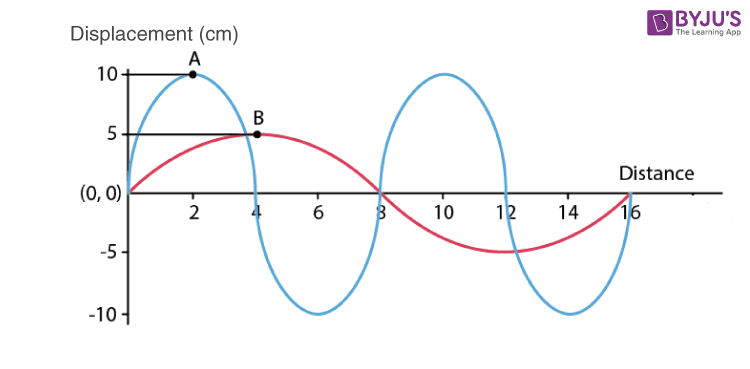Solution:

Amplitude is the maximum displacement from the mean position.

For A the maximum displacement = 10 cm and

For B the maximum displacement = 5 cm

The ratio of maximum amplitude is A1 / A2 = 10 / 5 = 2 / 1 = 2: 1

Wavelength of A = 8 cm

Wavelength of B = 16 cm

The ratio of wavelength is λ1 / λ2 = 8 / 16 = 1 / 2 = 1: 2

Exercise 7(b) page no: 163

Question: 1

What do you understand by the natural vibrations of a body? Give one example.

Solution:

Natural or free vibrations are the vibrations of a body in the absence of any external force on it.

For example:

Various strings are set in vibration each at its own natural frequency when we strike the keys of a piano.

Question: 2

What is meant by the natural frequency of vibrations of a body? Name one factor on which it depends?

Solution:

The natural frequency of vibration of the body occurs when each body capable of vibrating is set to vibrate freely, with a frequency f.

The natural frequency vibration of a body depends on the shape and size of the body.

Question: 3

(a) Draw a graph between displacement and time for a body executing the natural vibrations

(b) Where can a body execute the natural vibrations?

Solution:

The displacement time graph for the free vibrations is shown below for a body executing free vibrations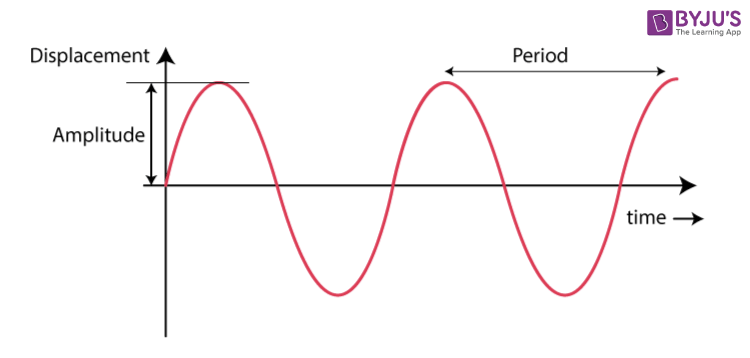The body executes natural vibrations when the oscillations of a body is set into vibrations by any external force and then left to itself.

Question: 4

State one condition for a body to execute the natural vibrations.

The presence of medium around the body offers some resistance due to which the amplitude of vibration continuously decreases and does not remain constant. Hence, for this reason, the natural vibrations of a body occur only in a vacuum.

Question: 5

(a) Name one factor on which the frequency of sound emitted due to vibration in an air column depends.

(b) How does the frequency depend on the factor stated in part (a)?

Solution:

(a) When the frequency of sound emitted due to vibration in an air column, it affects the length of the air column.

(b) The frequency decreases as the length of the air column increases. Hence we can say that they are inversely proportional to each other i.e f ∝ 1 / l

Question: 6

State one way of increasing the frequency of a note produced by an air column.

Solution:

By decreasing the length of the air column the frequency of the note produced in the air column can be increased.

Question: 7

State two ways of increasing the frequency of vibrations of a stretched string.

Solution:

The two ways of increasing the frequency of vibrations of a stretched string are

(i) By increasing the tension in the string

(ii) By decreasing the length of the string

Question: 8

How does the frequency of sound given by a stretched string depend on its (a) length, (b) tension?

Solution:

(a) Length: The frequency of sound is inversely proportional to the length of the string.

f ∝ 1 / l

(b) Tension: The frequency of sound is directly proportional to the square root of the tension in the string.

f ∝ √T

Question: 9

What adjustments would you make for tuning a stringed instrument for it to emit a note of a desired frequency?

Solution:

By varying the tension, we can get the desired frequency. Thus a stringent instrument is provided with the provision for adjusting the tension of the string.

Question: 10

The diagram below in Fig. shows three ways in which the string of an instrument can vibrate.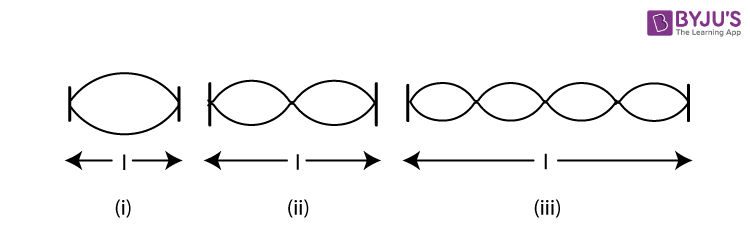(a)Which of the diagram shows the principal note?

(b)Which vibration has the frequency four times that of the first?

(c) Which vibration is of longest wavelength?

(d)What is the ratio of the frequency of vibrations in the diagram (i) and (ii)?

Solution:

(a) Diagram showing the principle note is (i)

(b) Diagram which has the frequency four times that of the first is (iii)

(c) Diagram having the longest wavelength is (i)

(d) The ratio of the frequency of vibrations in the diagram (i) and (ii) is 1: 2

Question: 11

Explain why strings of different thickness are provided on a stringed instrument.

Solution:

In order to produce different frequency sound waves, strings of different thickness are provided on a stringed instrument. This is because the natural frequency of vibration of a stretched string is inversely proportional to the radius of the string.

Question: 12

A blade, fixed at one end, is made to vibrate by pressing its other end and then releasing it. State one way in which the frequency of vibrations of the blade can be lowered.

Solution:

By increasing the length of the blade or by sticking a small weight on the blade at its free end, the frequency of vibrations of the blade is lowered.

Question: 13

How does the medium affect the amplitude of the natural vibrations of a body?

Solution:

The surrounding medium offers resistance to the motion, so the energy of the vibrating body continuously decreases due to which the amplitude of vibration decreases gradually.

Question: 14

What are the damped vibrations? How do they differ from free vibrations? Give one example of each.

Solution:

The damped vibration is the periodic vibrations of a body of decreasing amplitude in the presence of a resistive force.

When a body is made to vibrate in a medium, the amplitude of the vibrating body continuously decreases with time and ultimately the body stops vibrating.

For example: Slim branch of a tree makes damped vibrations when it is pulled and then released. The vibrations of a spring having a mass at its end, in the air are the damped vibrations.

Question: 15

The diagram in figure shows the displacement-time graph of a vibrating body.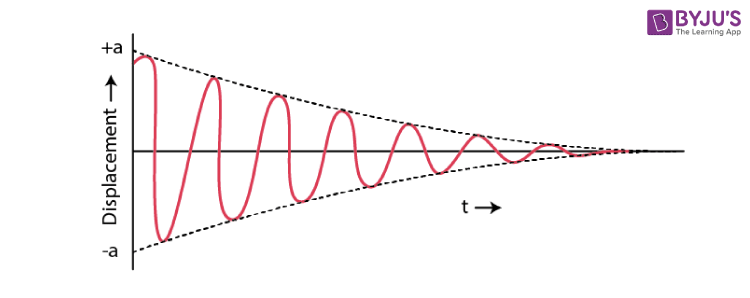(i) Name the kind of vibrations.

(ii) Give one example of such vibration.

(iii) Why is the amplitude of vibrations gradually decreasing?

(iv) What happens to the vibrations of the body after some time?

Solution:

(i) Damped vibrations

(ii) Example: A tuning fork when stroked on a rubber pad, executes the damped vibrations in air.

(iii) The amplitude of vibrations decreases due to the frictional force. The energy of the vibrating body continuously dissipates in doing work against the force of friction. Hence, its amplitude gradually decreases.

(iv) After some time it stops vibrating when it has lost all its energy.

Question: 16

A tuning fork is set into vibrations in the air. Name the kind of vibrations it executes.

Solution:

A tuning fork executes damped vibrations in air

Question: 17

Draw a sketch showing the displacement of a body executing damped vibrations, against time.

Solution:

The displacement time graph of damped vibrations shown below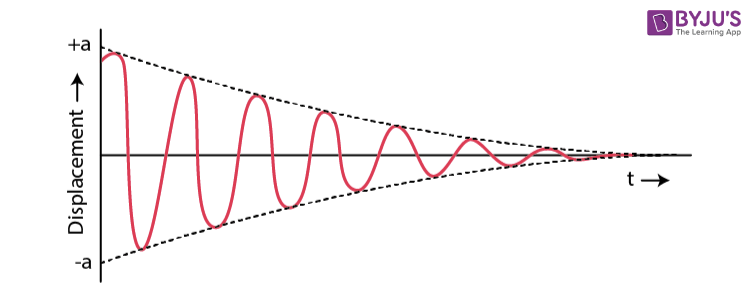Question: 18

What are the forced vibrations? Give one example to illustrate your answer.

Solution:

Forced vibrations are the vibrations of a body which take place under the influence of an external periodic force acting on it. When an artist plays a guitar he forces the strings of the guitar to execute forced vibrations.

Question: 19

On keeping the stem of a vibrating tuning fork on the surface of a table, a loud sound is heard. Give reason.

Solution:

The tuning fork produces the forced vibrations in the tabletop when the stem of a vibrating tuning fork is pressed against the top of a table. We hear a loud sound called resonance when the frequency of the periodic force applied on a body is equal to the natural frequency of that body. Hence because of this resonance, a loud sound is heard on keeping the stem of a vibrating tuning fork on the surface of a table.

Question: 20

State two differences between the natural and forced vibrations

Solution:

(i) Free vibrations occur when a body is displaced from its equilibrium position and released while the forced vibration is produced by an external periodic force of any frequency.

(ii) Amplitude in free vibrations decreases with time and in forced vibration amplitude is small but remain constant as the force is acting.

Question: 21

What is meant by resonance? Describe a simple experiment to illustrate the phenomenon of resonance and explain it.

Solution:

When the frequency of the externally applied periodic force on a body is equal to its natural frequency, with an increased amplitude the body readily begins to vibrate. This phenomenon is known as ‘resonance’ and is a special case of forced vibrations.

Mount two identical tuning forks A and B, both having a same frequency on two separate sound boxes such that their open ends face each other as shown in below figure.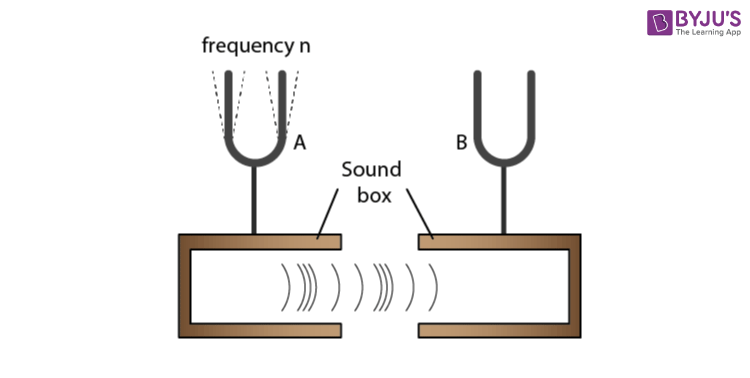The prong A of one of the tuning forks starts vibrating when it is struck on a rubber pad. On putting this tuning fork A on its soundbox, the tuning fork B also starts vibrating and a loud sound is heard. It is due to the resonance, the vibrations are produced in B.

Question: 22

State the condition for the resonance to occur.

Solution:

Condition for resonance:

When the applied force causes forced vibration in the body and the frequency of the applied force is exactly equal to the natural frequency of the vibrating body, then the phenomenon called resonance occurs.

Question: 23

Complete the following sentence:

Resonance is a special case of ______ vibrations, when the frequency of the driving force is _______ natural frequency of the driven body.

Solution:

Resonance is a special case of forced vibrations, when the frequency of the driving force is equal to the natural frequency of the driven body.

Question: 24

State two differences between the forced and resonant vibrations

Solution:

 Forced vibrations Resonant vibrations The amplitude of vibration is small The amplitude of vibration is very large The vibrations of the body are not in phase with the external periodic force. The vibrations of the body are in phase with the external periodic force.

Question: 25

Why is a loud sound is heard at resonance?

Solution:

The body vibrates with a large amplitude at resonance, sends forth a large amount of energy in the medium. Thus conveying more energy to the ear, a loud sound is heard.

Question: 26

Figure shows two tuning forks A and B of the same frequency mounted on two separate sound boxes with their open ends facing each other. The fork A is set into vibration. (a) Describe your observation. (b) State the principle illustrated by this experiment.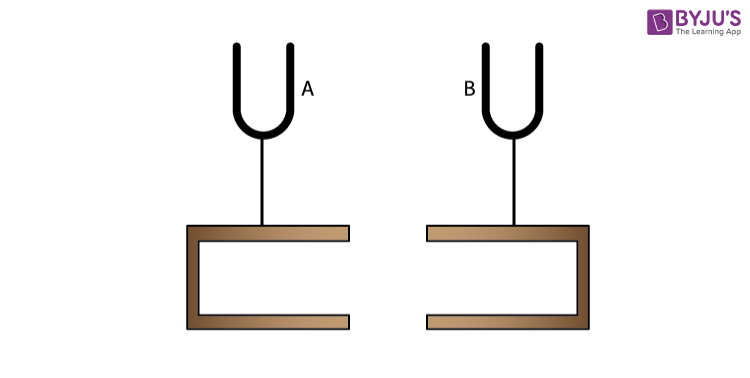Solution:

Forced vibrations are produced by the vibrating tuning fork A in the air column of its soundbox. Due to the large surface area of air in the soundbox, the vibrations are of large amplitude. They are communicated to the soundbox of the fork B. The air column of B starts vibrating with the frequency of fork A. Since the frequency of these vibrations is same as the natural frequency of the fork B, the fork B picks up these vibrations and starts vibrating under resonance.

On putting the tuning fork A to vibrate, the other tuning fork B also starts vibrating. Due to the resonance, the vibrations are produced in the second tuning fork B.

Question: 27

In figure A, B, C and D are the four pendulums suspended from the same elastic string XY. The lengths of pendulum A and D are equal, while the length of pendulum B is shorter and of the pendulum C is longer. The pendulum A is set into vibrations.

(b) Give reason for your observation in part (a).

(c) What type of vibrations take place in pendulums B and C?

(d) Give reason for the answer in part (c)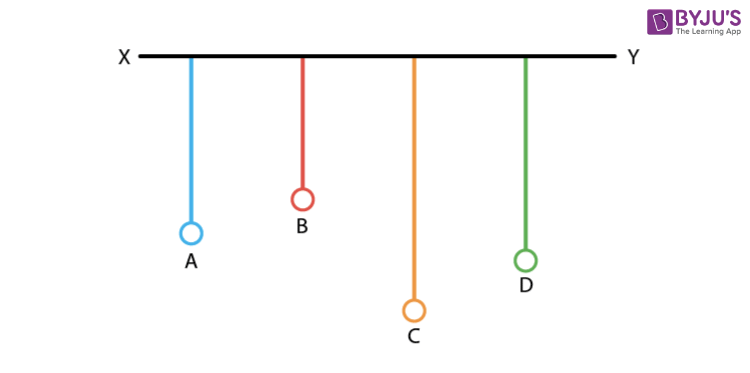Solution:

Set the pendulum A, normal to its length into vibration by displacing it to one side. It is observed that pendulum D starts vibrating initially with a small amplitude and ultimately it acquires the same amplitude as the pendulum A had initially. Since the total energy is constant, as the amplitude of the pendulum D becomes maximum, the amplitude of the pendulum A becomes minimum. After some time the amplitude of the pendulum D will decrease and the amplitude of A increases. Only between the pendulums A and D, the exchange of energy takes place as their natural frequencies are same. Also, the pendulums B and C vibrate with very small amplitudes.

The vibrations produced in pendulum A are communicated as forced vibrations to the other pendulums B, C and D through XY. The pendulum D comes in the state of resonance while the pendulums B and C remain in the state of forced vibrations.

Question: 28

A vibrating tuning fork, held over an air column of a given length with its one end closed, produces a loud audible sound. Name the phenomenon responsible for it and explain the observation.

Solution:

The phenomenon responsible for producing a loud audible sound when a vibrating tuning fork, held over an air column of a given length with its one end is known as resonance. Forced vibrations in the air column are caused by the vibrating tuning fork. A loud sound is heard for a certain length of air column. This happens when the frequency of the air column becomes equal to the frequency of the tuning fork.

Question: 29

In figure A, B, C and D represent the test tubes each of height 20 cm which are filled with water up to heights of 12 cm, 14 cm, 16 cm and 18 cm respectively. If a vibrating tuning fork is placed over the mouth of test tube D, a loud sound is heard.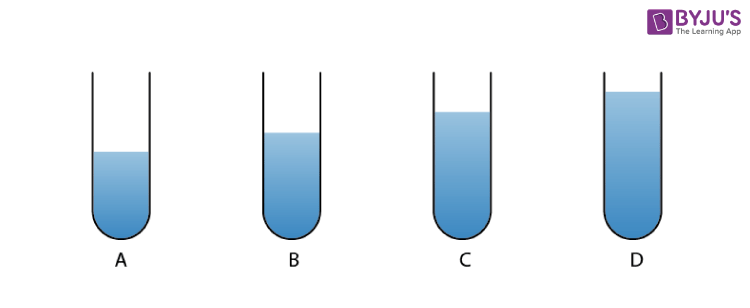(a) Describe the observations with the tubes A, B and C when the vibrating tuning fork is placed over the mouth of these tubes.

(b) Give the reason for your observation in each tube.

(c) State the principle illustrated by the above experiment.

Solution:

(a) No loud sound is heard with the tubes A and C, but a loud sound is heard with the tube B.

(b) Frequency of air column in tube D is equal to the frequency of tuning fork. Resonance occurs with the air column in tube B whereas no resonance occurs with the air column of tubes A and C. The frequency vibrations of the air column in tube B is same as the frequency of vibrations of the air column in tube D because the length of the air column in tube D is 20 – 18 = 2 cm and that in tube B is 20 – 14 = 6 cm. But the frequency of vibrations of the air column in tubes A and C is not equal to the frequency vibrations of air column in tube B

(c) Resonance occurs when the frequency of vibrations of the air column is equal to the frequency of the vibrating tuning fork.

Question: 30

When a troop crosses a suspension bridge, the soldiers are asked to break their steps. Why?

Solution:

While the soldiers march in steps, all the separate periodic forces exerted by them are in the same phase and hence in the bridge forced vibrations of a particular frequency are produced. If the natural frequency of the bridge happens to be equal to the frequency of the steps, due to resonance the bridge will vibrate with large amplitude and suspension bridge could crumble. Thus when a troop crosses a suspension bridge, the soldiers are asked to break steps.

Question: 31

Why are the stringed instruments like guitar provided with a hollow soundbox?

Solution:

The soundbox is constructed in such a way that the column of air inside it, has a natural frequency which is same as that of the string stretched on it, so that when the strings vibrate, the air column inside the box is set into forced vibrations. Soundbox sets a large volume of air into vibration due to its large area, the frequency of which is the same as that of the string. Hence, a loud sound is produced due to resonance.

Question: 32

How do you tune your radio set to a particular station? Name the phenomenon involved in doing so and define it.

Solution:

To tune a radio receiver, we only adjust the values of the electronic components to produce vibrations of frequency equal to that of the radio waves which we want to receive. When both the frequencies match, the energy of the signal of that particular frequency is received from the incoming waves due to resonance. The signal received is then amplified in the receiver set.

This phenomenon associated resonance and is a special case of forced vibrations. When the frequency of an externally applied periodic force on a body is equal to its natural frequency, the body quickly begins to vibrate with an increased amplitude. This phenomenon is called resonance.

MULTIPLE CHOICE TYPE

Question: 1

A wire stretched between two fixed supports is plucked exactly in the middle and then released. It executes (neglect the resistance of the medium):

(a) Resonant vibrations

(b) natural vibrations

(c) Damped vibrations

(d) Forced vibrations

Solution:

A wire stretched between the two fixed supports, is plucked exactly in the middle and then released. It executes natural vibrations.

Question: 2

When a body vibrates under a periodic force, the vibrations of the body are:

(a) natural vibrations

(b) Damped vibrations

(c) Forced vibration

(d) Resonant vibrations

Solution:

When a body vibrates under a periodic force, the vibrations of the body are forced vibrations

Question: 3

A tuning fork of frequency 256 Hz will resonate with another tuning fork of frequency:

a. 128 Hz

b. 256 Hz

c. 384 Hz

d. 512 Hz

Solution:

A tuning fork of frequency 256 Hz will resonate with another tuning fork of frequency 256 Hz

Exercise 7(c) page no: 172

Question: 1

Name three characteristics of a musical sound.

Solution:

The three characteristics of sound are

(i) Loudness

(ii) Pitch or shrillness

(iii) Quality or timber

Question: 2

(a)Which of the following quantity determines the loudness of a sound wave?

(i)Wavelength

(ii) frequency and

(iii) amplitude

(b)How is loudness related to the quantity mentioned in the above part (a)

Solution:

(a) The amplitude of the wave determines the loudness of a sound wave. A larger amplitude means a loud sound while a smaller amplitude means a soft sound.

(b) Loudness is proportional to the square of the amplitude.

Question: 3

If the amplitude of a wave is doubled, what will be the effect on its loudness?

Solution:

Since the loudness of sound is directly proportional to the square of the amplitude. Thus if the amplitude of the wave is doubled then the sound will become 4 times louder.

Question: 4

Two waves of the same pitch have amplitudes in the ratio 1:3. What will be the ratio of their (i) loudness (ii) frequencies?

Solution:

(i) Loudness is proportional to the square of the amplitude

∴ Ratio of loudness = 1: 9

(ii) If pitch is unchanged, then the frequency remains the same.

∴ Ratio of frequencies = 1: 1

Question: 5

How does the wave pattern of a loud note differ from the soft note? Draw a diagram.

Solution: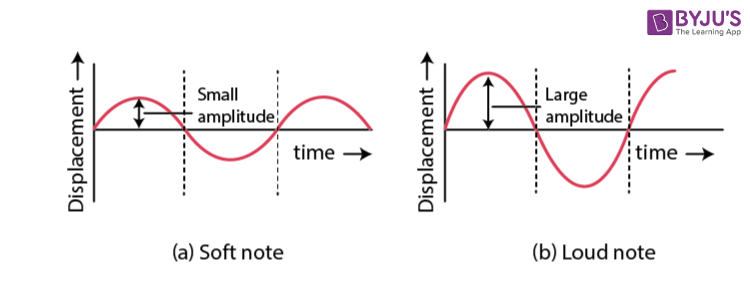Question: 6

Name the unit in which the loudness of sound is measured?

Solution:

The loudness of sound is measured in units known as decibels

Question: 7

Why is the loudness of the sound heard by a plucked wire increased when it is mounted on a soundboard?

Solution:

This is because, the board provides almost a large area and forces a large volume of air to vibrate and hence increases the sound energy reaching our ears.

Question: 8

Define the term intensity of a sound wave. State the unit in which it is measured.

Solution:

The intensity of the sound wave at a point of the medium is the amount of sound energy passing per second normally through a unit area at that points. The unit of intensity of the sound wave is watt per metre2 (W m-2)

Question: 9

How is the loudness of sound related to the intensity of wave producing it?

Solution:

Relationship between loudness L and intensity I is given by

L = K log10 I

where K is constant of proportionality

Question: 10

Comment on the statement ‘loudness of sound is a subjective nature, while intensity is an objective nature’

Solution:

The intensity of a sound wave at a point of the medium is the amount of sound energy passing per second normally through a unit area at that point.

The loudness of a sound depends on the intensity i.e the energy conveyed by the sound wave near the eardrum of the listener. Loudness also depends on the sensitivity of the ears of the listener. Thus the loudness of sound of a given intensity may differ from listener to listener. Further, two sounds of the same intensity but of different frequencies may differ in loudness even to the same listener. This is because the sensitivity of ears is different for different frequencies. Therefore the loudness has a subjective nature while intensity, being a measurable quantity, has an objective nature.

Question: 11

State three factors which affect the loudness of sound heard by a listener.

Solution:

The loudness of sound heard by a listener depends on the following factors

(i) Loudness is proportional to the square of the amplitude

(ii) Loudness is inversely proportional to the square of the distance

(iii) Loudness depends on the surface area of the vibrating body.

Question: 12

The bells of a temple are big in size. Why?

Solution:

According to the study of bells, larger bells provide less resonant frequency. It is essential that the frequency of the sound is less for a sound wave to travel long distances. Also, it is observed that thicker the body of a bell, the quality of sound will be richer. Hence the bells of a temple are big in size.

Question: 13

Name the unit used to measure the sound level.

Solution:

The unit to measure the sound level is decibel

Question: 14

What is the safe limit of sound level in dB for our ears?

Solution:

The safe limit of sound level in dB for our ears is up to 120 dB

Question: 15

What is meant by noise pollution? Name one source of sound causing noise pollution.

Solution:

The disturbance produced due to undesirable loud and harsh sound of the level above 120 dB from the various sources such as a loudspeaker, moving vehicles etc in the environment is called noise pollution.

Question: 16

What determines the pitch of sound?

Solution:

Pitch of a note depends on its frequency. Two notes with same amplitude sounded on the same instrument, will differ in pitch when their vibrations are of different frequencies.

Question: 17

Name the subjective property of sound related to its frequency.

Solution:

Pitch is the subjective property of sound related to its frequency

Question: 18

Name and define the characteristic which enables one to distinguish two sounds of same loudness, but of different frequencies given by the same instrument.

Solution:

The characteristic by which two sounds of same loudness, but of different frequencies given by the same instrument can be distinguished known as ‘pitch’.

Question: 19

Draw a diagram to show the wave pattern of high pitch note and a low pitch note, but of the same loudness.

Solution:

The diagram for high pitch note is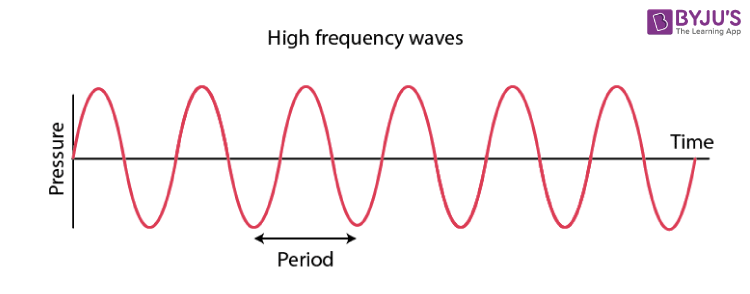The diagram for low pitch note is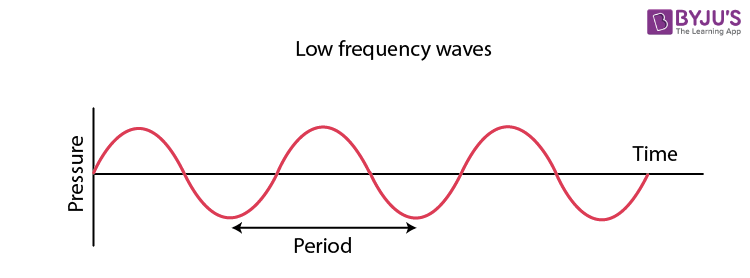Question: 20

How is it possible to detect the filling of a bottle under a water tap by hearing the sound at a distance?

Solution:

The length of air column decreases, as the water level in a bottle kept under a water tap rises. Thus the frequency of sound produced increases. Hence one can get the idea of water level in the bottle by hearing sound from a distance.

Question: 21

The frequencies of notes given by flute, guitar and trumpet are respectively 400 Hz, 200 Hz and 500 Hz. Which one of these has the highest pitch?

Solution:

The frequency of trumpet is highest. So the trumpet has the highest pitch.

Question: 22

Complete the following sentences:

(a)The pitch of sound increases, if its frequency ___________.

(b)If the amplitude of the sound is halved, its intensity becomes __________.

Solution:

(a) The pitch of sound increases, if its frequency increases.

(b) If the amplitude of the sound is halved, its intensity becomes one-fourth.

Question: 23

The diagram below shows three different modes of vibration P, Q and R of the same string of a given length.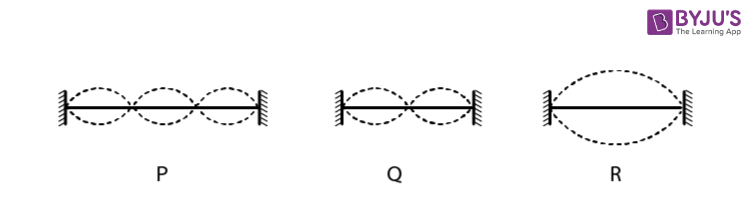(a) Which vibration will produce a louder sound and why?

(b) Which vibration will produce sound of maximum shrillness (or pitch) and why?

(c) What is the ratio of the wavelength of vibrations P and R?

Solution:

(a) The amplitude of R is maximum. So, R will produce a louder sound

(b) The frequency of P is maximum. So, P will produce a maximum shrillness.

(c) The ratio of wavelength of vibrations P and R is

λp: λR = 1: 3

Question: 24

Name the characteristic which enables one to distinguish the sound of two musical instruments even if they are of the same pitch and same loudness.

Solution:

The characteristic which enables one to distinguish the sound of two musical instruments even if they are of the same pitch and same loudness is quality or timbre of the sound.

Question: 25

How does the two sounds of the same loudness and same pitch produced by different instruments differ? Draw diagrams to illustrate your answer.

Solution:

The two sounds of the same loudness and same pitch produced by different instruments differ because of their different waveforms.

The waveforms depend on the number of the subsidiary notes and their relative amplitude along with the principal note.

The below diagram shows the wave patterns of two sounds of the same loudness and same frequency but of different waveforms. One is a sine wave and the other is a triangular wave.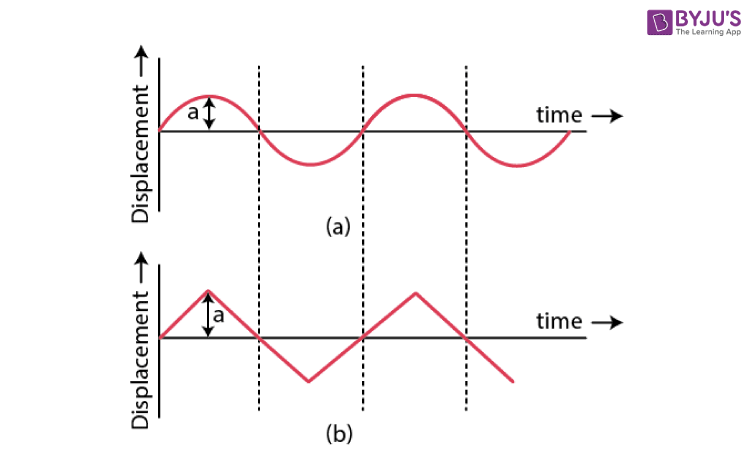Question: 26

Two identical guitars are played by two persons to give notes of the same loudness and pitch. Will they differ in quality? Give a reason for your answer.

Solution:

As the guitars are identical, they will have a similar waveform and hence, the similar quality.

Question: 27

Two musical notes of the same pitch and same loudness are played on two different instruments. Their wave patterns are as shown in the diagram below.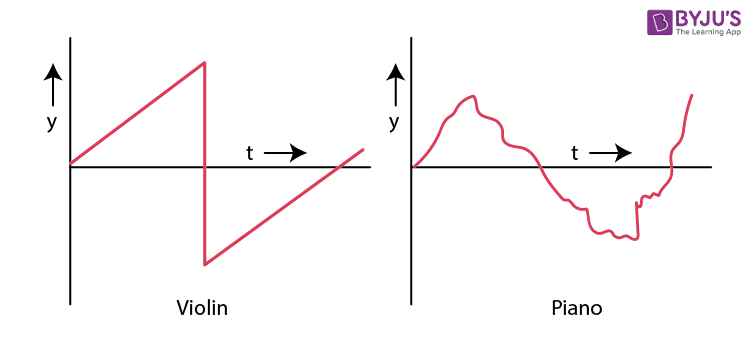Explain why the wave patterns are different.

Solution:

Different instruments produce different subsidiary notes. A note played on one instrument has a large number of subsidiary notes, while the same note played on other instrument contains only a few subsidiary notes. Hence, they have different waveforms.

Question: 28

Which characteristic of sound makes it possible to recognise a person by his voice without seeing him?

Solution:

The quality or timbre of a sound is the characteristic which enables us to recognise a person by his voice even without seeing him.

Question: 29

State the factor that determines

(i) the pitch of a note,

(ii) the loudness of the sound heard, and

(iii) the quality of the note.

Solution:

(i) Frequency determines the pitch of a note.

(ii) Amplitude determines the loudness of the sound heard

(iii) Waveforms determines the quality of the note

Question: 30

Name the characteristic of the sound affected due to a large in its (i) amplitude, (ii) waveform, and (iii) frequency

Solution:

(i) The characteristic of the sound affected due to large in its amplitude is loudness

(ii) The characteristic of the sound affected due to large in its waveform is quality

(iii) The characteristic of the sound affected due to large in its frequency is pitch

Question: 31

The sketches I to IV in figure show sound waves, all formed in the same time interval.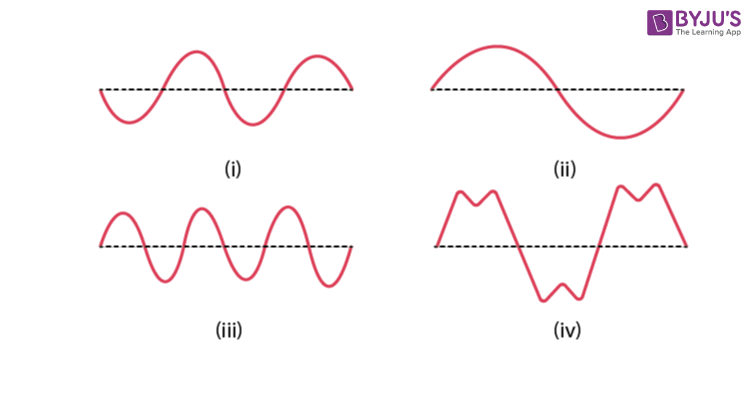Which diagram shows

(i) a note from a musical instrument,

(ii) a soft (or feeble) note,

(iii) a bass (or low frequency) note.

Solution:

(i) Figure IV is a note from a musical instrument

(ii) Figure I is a soft (or feeble) note

(iii) Figure II is a bass (or low frequency) note

Question: 32

Shows the wave patterns of three sounds A, B and C. Name the characteristic of sound which is same between (i) A and B, (ii) B and C, and (iii) C and A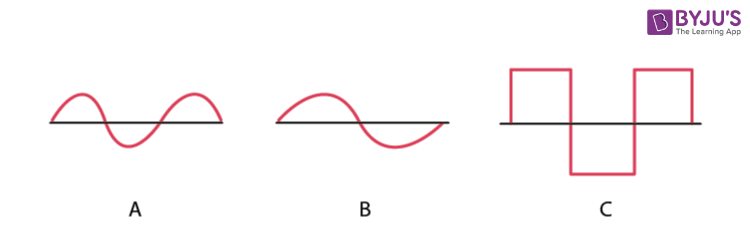Solution:

(i) Both A and B have the same amplitude and waveform. Thus loudness and quality is the same.

(ii) In figures B and C, neither amplitude nor waveform is the same. Therefore no characteristic is same

(iii) In figures C and A, frequency of both the sound is the same and hence, the pitch is the same

Question: 33

A microphone is connected to the Y-input of a C.R.O. Three different sounds are made in turn in front of the microphone. Their traces (a), (b) and (c) produced on the screen are shown in figure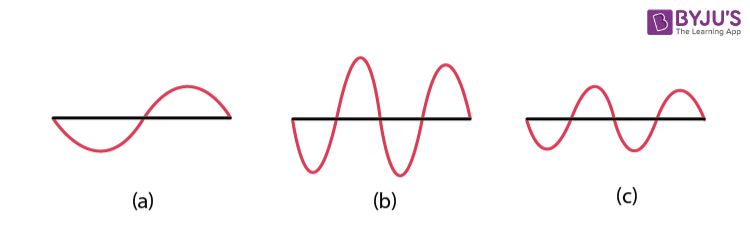(i) Which trace is due to the loudest sound? Give the reason for your answer.

(ii) Which trace is due to the sound with the lowest pitch? Explain your answer.

Solution:

(i) Figure (b) is due to the loudest sound because amplitude is largest

(ii) Figure (a) is due to the sound with the lowest pitch because frequency is the lowest

Question: 34

In what respect does the wave pattern of a noise and music differ? Draw diagram to explain your answer.

Solution:

The wave pattern of a noise is irregular while the wave pattern is regular in music. The below figure shows the waveforms of a music and a noise.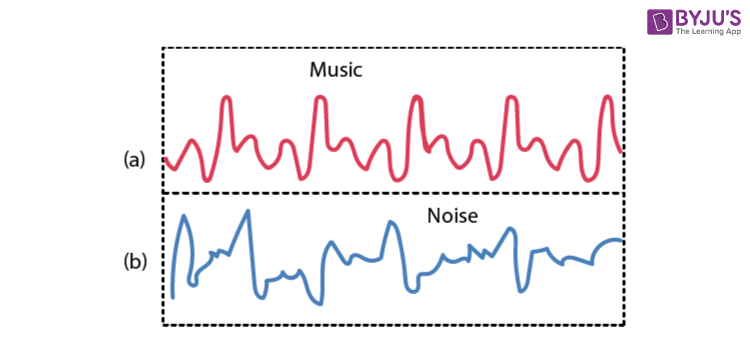Question: 35

State one difference between a musical note and a noise.

Solution:

 Music Noise It is regular, smooth and pleasant to the ears It is harsh, discordant and unpleasant to the ear The sound level is low (between 10 dB to 30 dB) The sound level is high (above 120 dB)

MULTIPLE CHOICE TYPE

Question: 1

By reducing the amplitude of the sound wave, its:

(a) pitch increases

(b) loudness decreases

(c) loudness increases

(d) pitch decreases

Solution:

If the amplitude of the sound wave is reduced, its loudness decreases

Question: 2

Two sounds of same loudness and same pitch produced by the two different instruments differ in their

(a) amplitudes

(b) frequencies

(c) waveforms

(d) all the above

Solution:

Two sounds of same loudness and same pitch produced by the two different instruments differ in their waveforms

Question: 3

Two sounds A and B are of same amplitudes, same waveforms but of frequencies f and 2f respectively. Then:

(a) B differ in quality from A

(b) B is grave, A is shrill

(c) B is shrill, A is grave

(d) B is louder than A

Solution:

Two sounds A and B are of same amplitudes, same waveforms but of frequencies f and 2f respectively. Then B is shrill, A is grave.

Selina Solutions Concise Physics Class 10 Chapter 7 Sound talks about the characteristics of sound and its different effects. The concepts covered in this chapter are sound waves, reflection of sound waves, echo, determination of the speed of sound by the method of echo, uses of echoes. Students who want to improve their performance in their academics are suggested to practice Selina Solutions.

List of subtopics covered in Selina Solutions Concise Physics Class 10 Chapter 7 Sound

 Number Subtopic 7.1 Sound 7.2 Reflection of Sound waves 7.3 Echo 7.4 Determination of speed of Sound by the method of echo 7.5 Uses of echoes 7.6 Natural vibrations 7.7 Damped vibrations 7.8 Forced vibrations 7.9 Resonance (a special case of forced vibrations) 7.10 Some examples of resonance 7.11 Characteristics of Sound 7.12 Music and noise

List of Exercises

 Name of the exercise Page number 7(A) 154 7(B) 163 7(C) 172

### Key Features of Selina Solutions Concise Physics Class 10 Chapter 7 Sound

• Selina Solutions are crafted by our subject experts in a simple language, which help the students understand the chapter easily
• Students obtain good marks by practising the solutions effectively
• These solutions help the students to quickly grasp the concepts of the chapter
• Selina Solutions help students to get a good foundation in Physics

The given solutions are as per the 2019-20 Concise Selina textbook. The Selina Solutions for the academic year 2023-24 will be updated soon.